Anzeige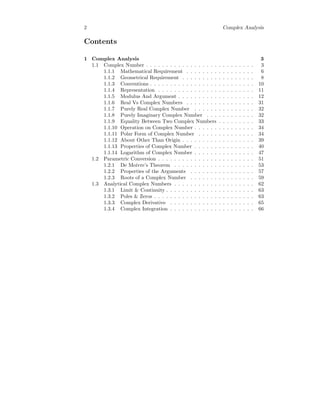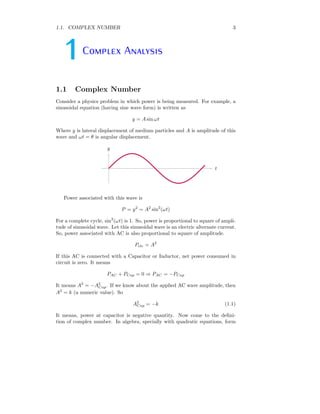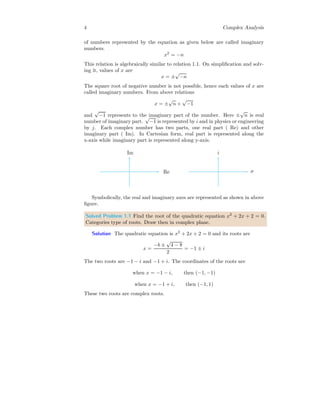Anzeige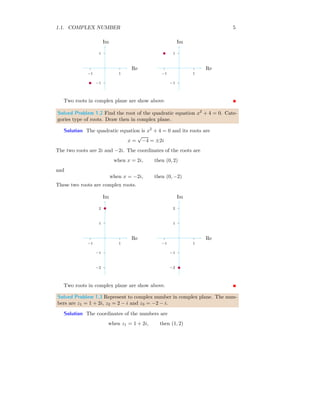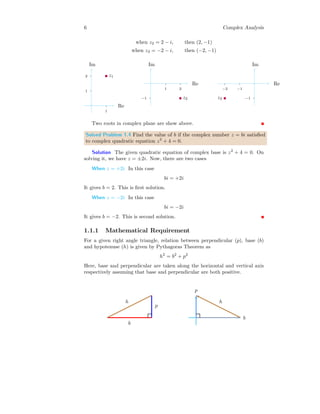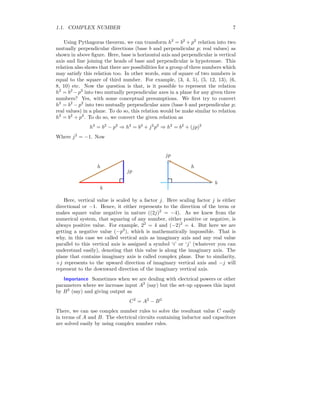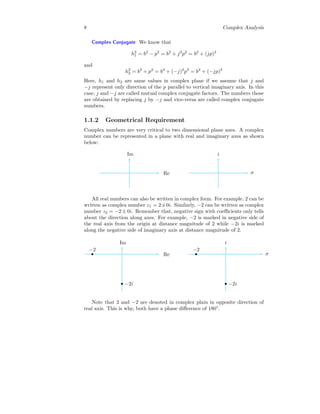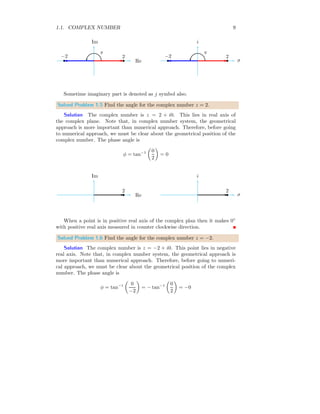Anzeige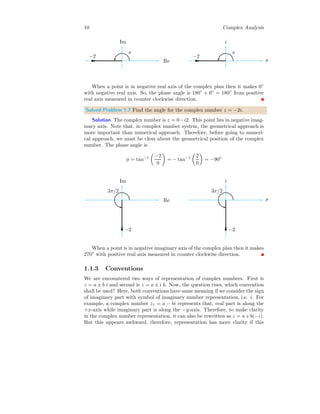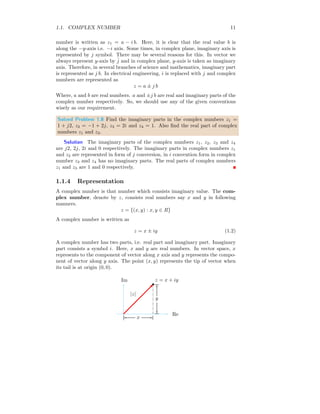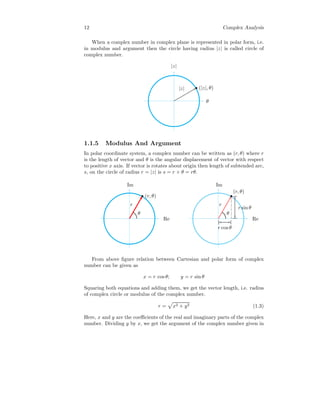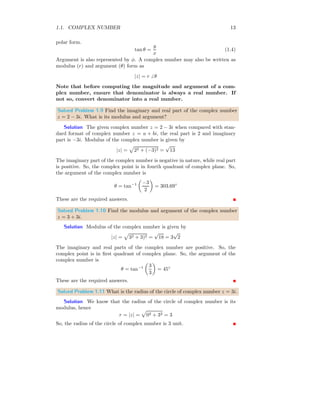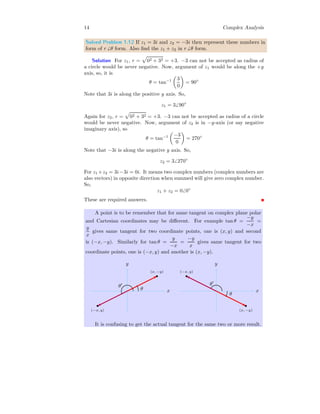Anzeige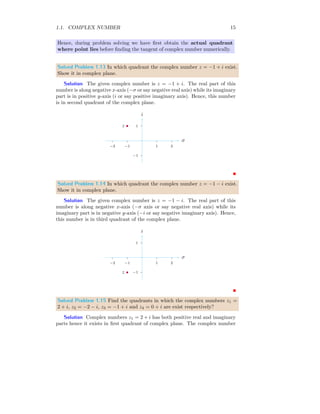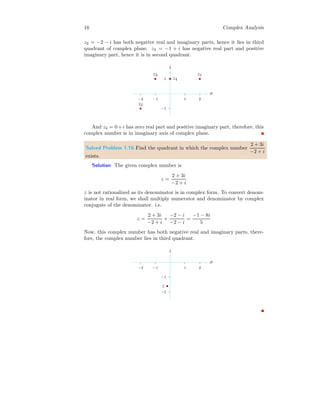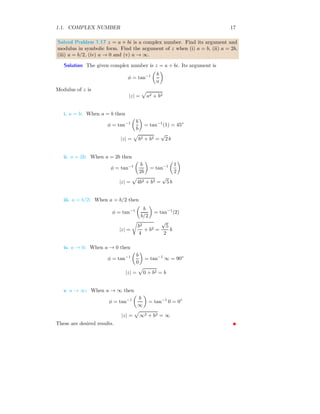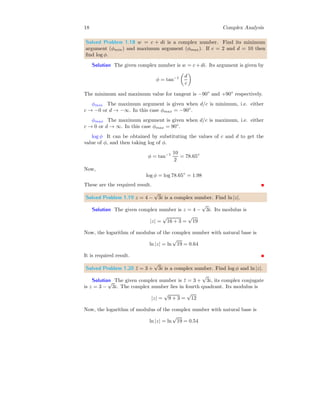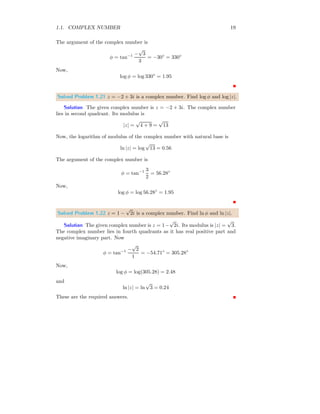Anzeige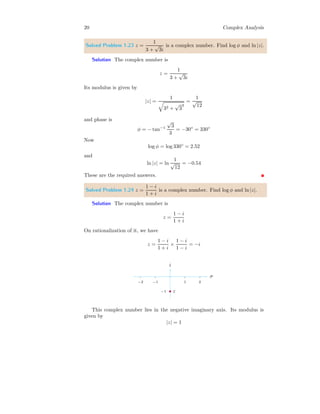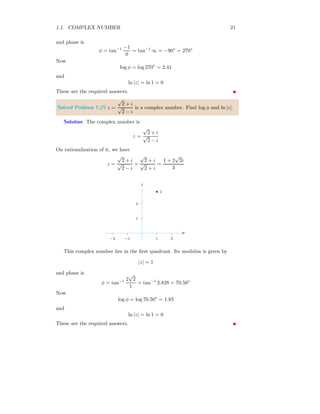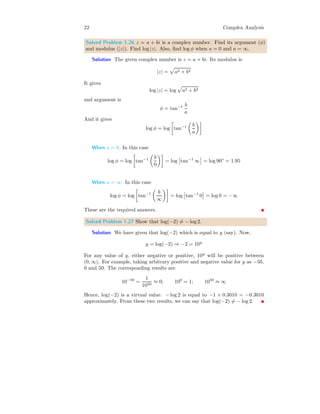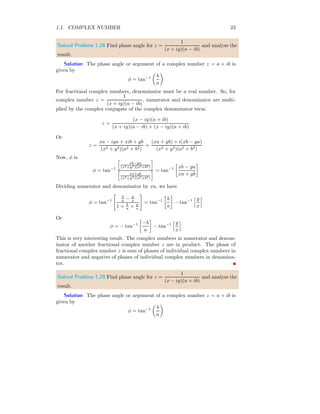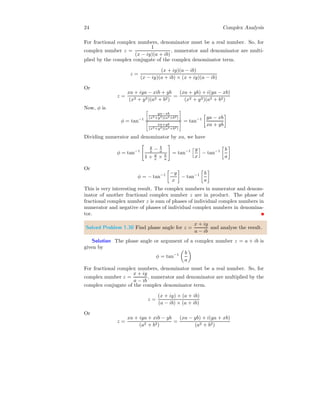Anzeige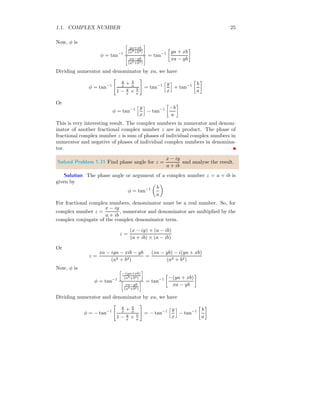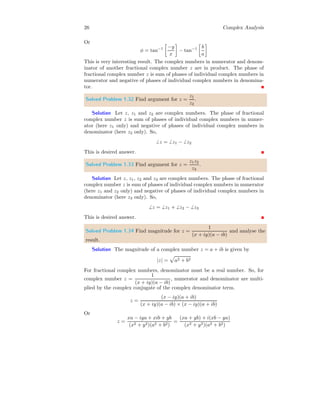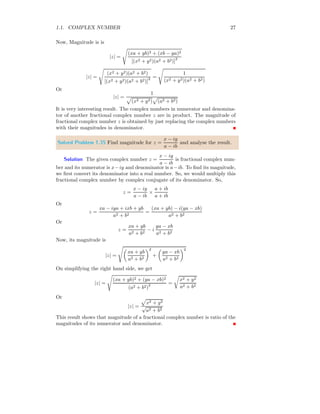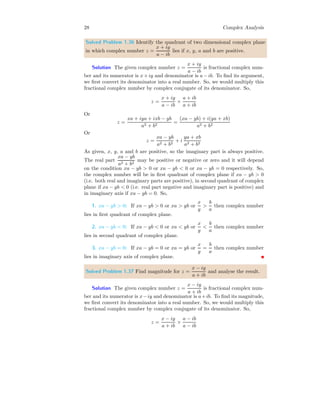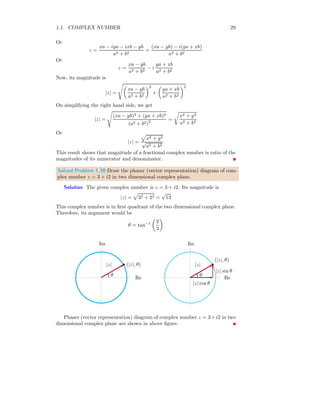Anzeige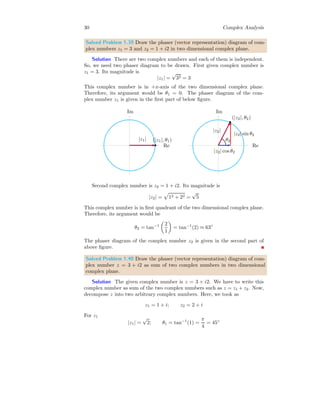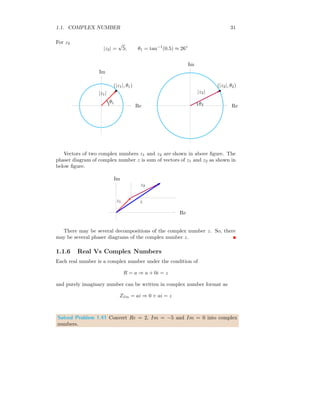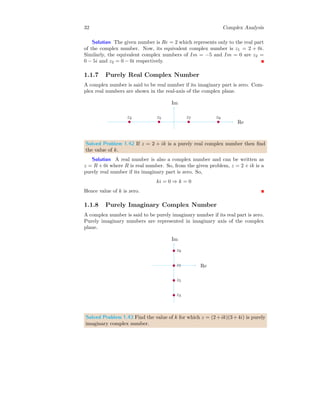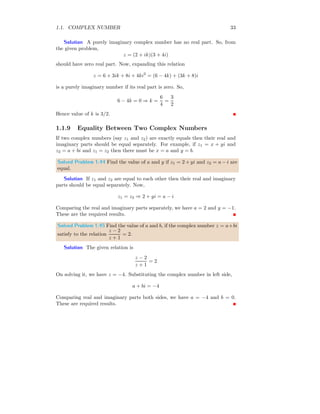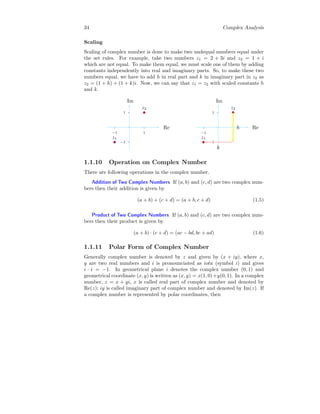Anzeige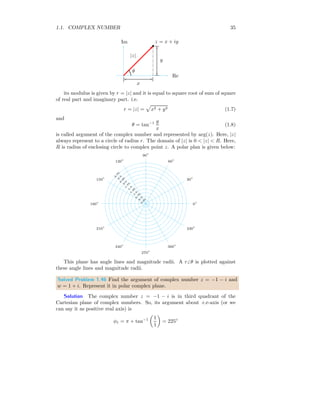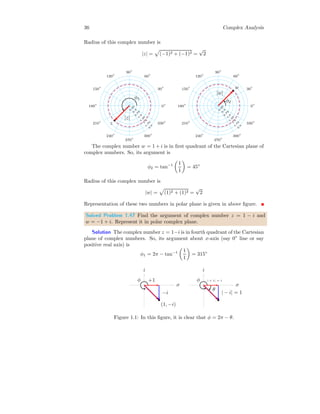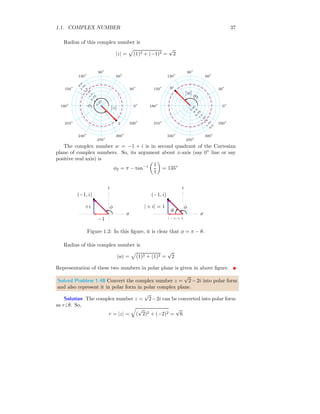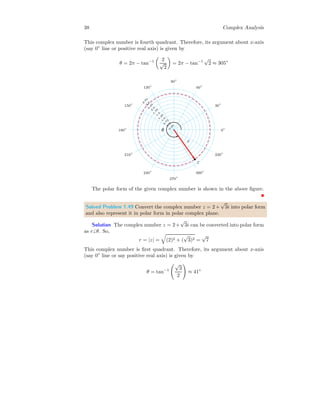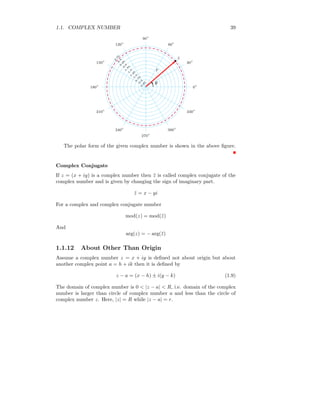Anzeige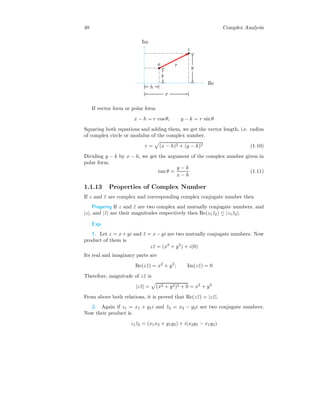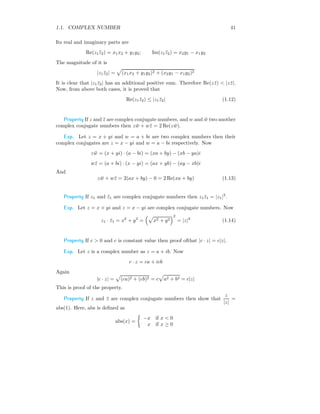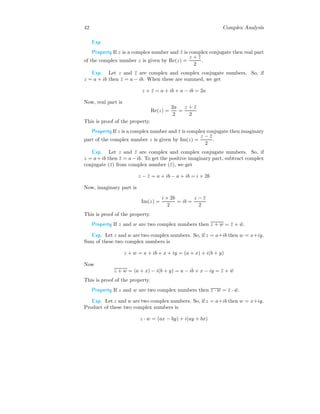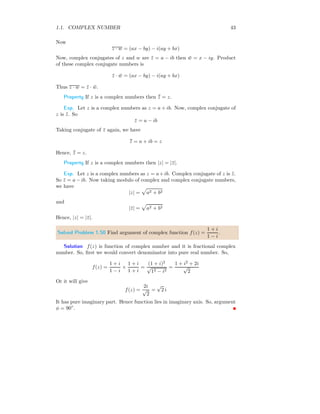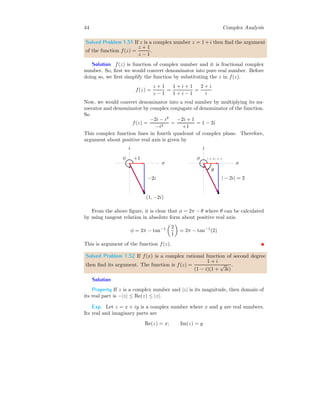Anzeige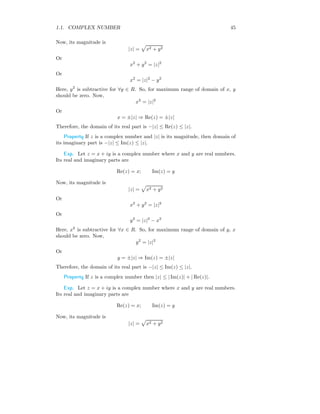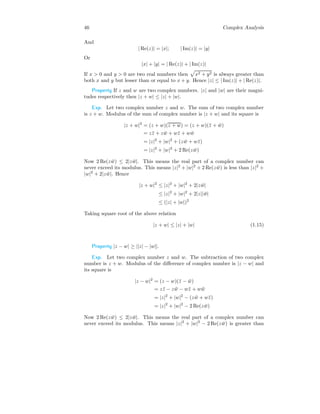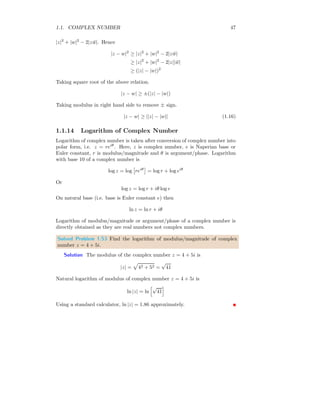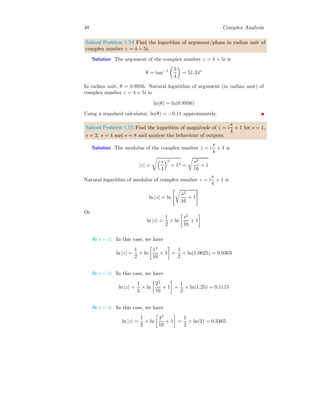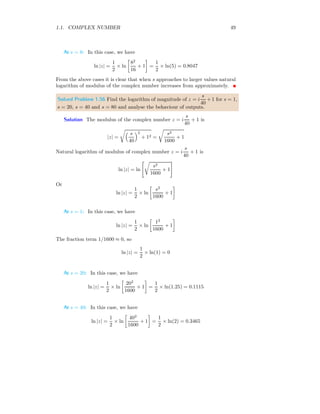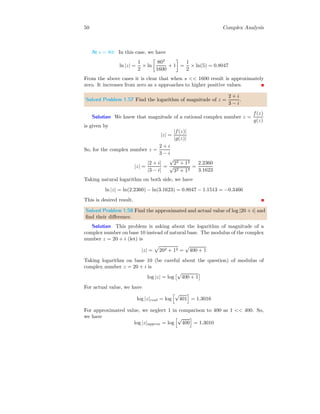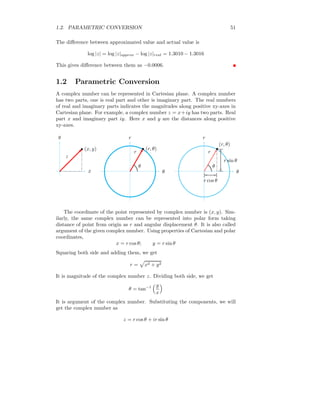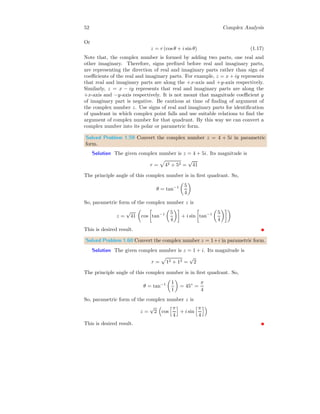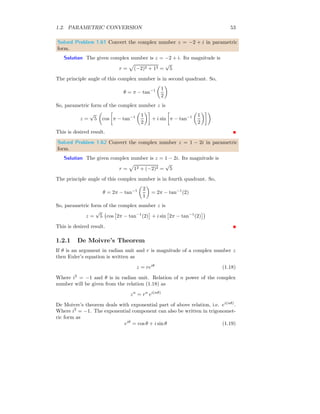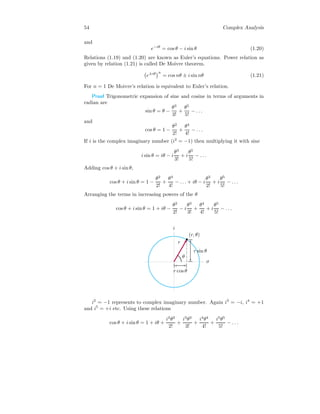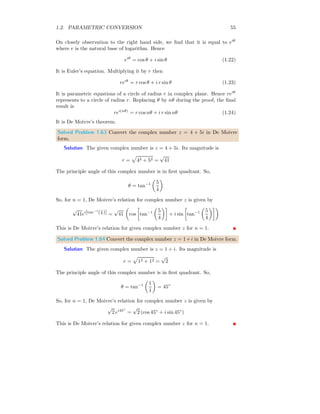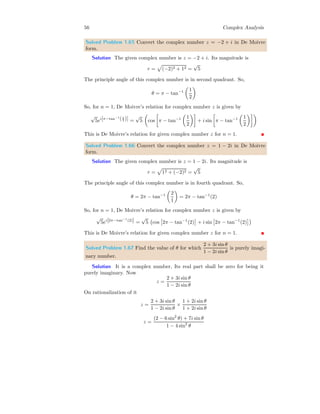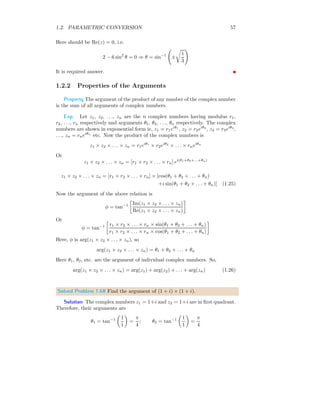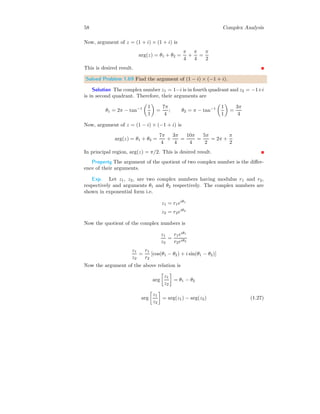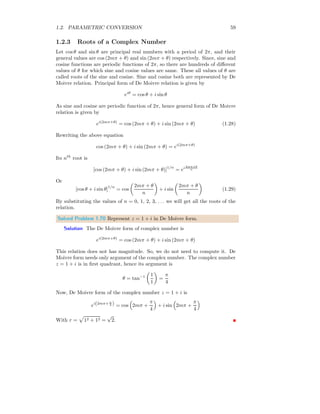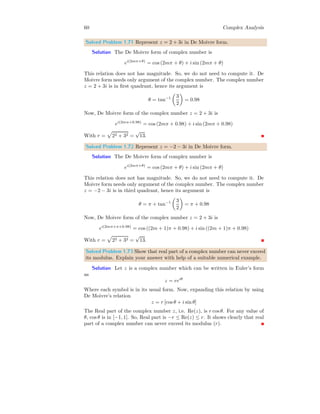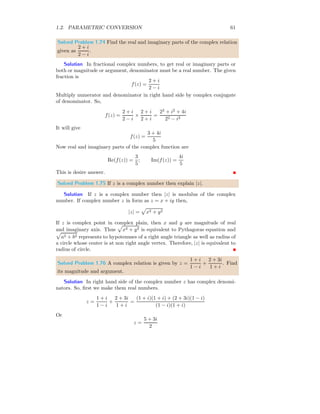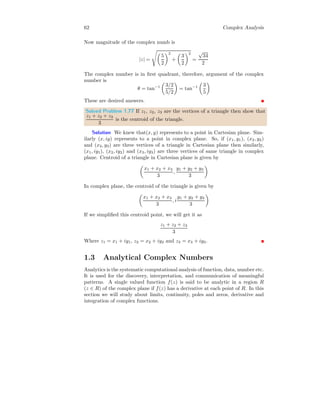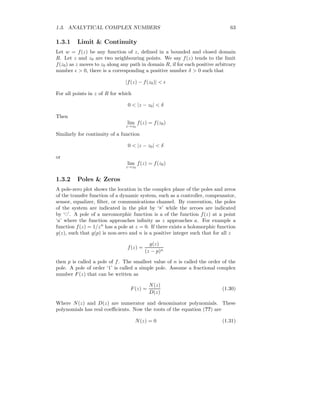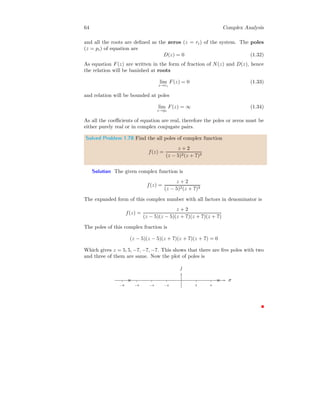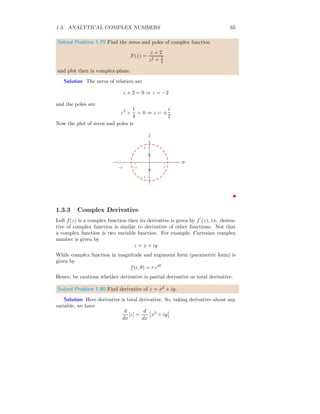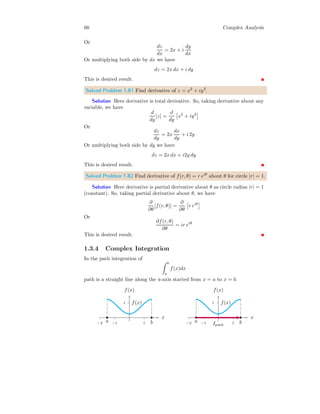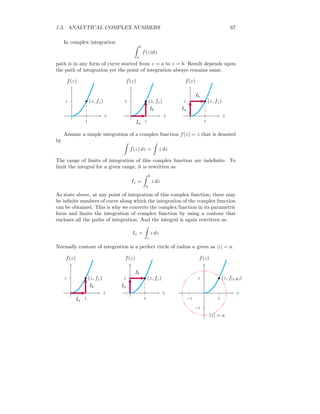Nächste SlideShareMETHOD OF JACOBI
1 von 67
Anzeige

### Complex Numbers

1. 1 COMPLEX NUMBERS NOTES FOR INTRODUCTION Arun Umrao https://sites.google.com/view/arunumrao DRAFT COPY - GPL LICENSING
2. 2 Complex Analysis Contents 1 Complex Analysis 3 1.1 Complex Number . . . . . . . . . . . . . . . . . . . . . . . . . . . 3 1.1.1 Mathematical Requirement . . . . . . . . . . . . . . . . . 6 1.1.2 Geometrical Requirement . . . . . . . . . . . . . . . . . . 8 1.1.3 Conventions . . . . . . . . . . . . . . . . . . . . . . . . . . 10 1.1.4 Representation . . . . . . . . . . . . . . . . . . . . . . . . 11 1.1.5 Modulus And Argument . . . . . . . . . . . . . . . . . . . 12 1.1.6 Real Vs Complex Numbers . . . . . . . . . . . . . . . . . 31 1.1.7 Purely Real Complex Number . . . . . . . . . . . . . . . 32 1.1.8 Purely Imaginary Complex Number . . . . . . . . . . . . 32 1.1.9 Equality Between Two Complex Numbers . . . . . . . . . 33 1.1.10 Operation on Complex Number . . . . . . . . . . . . . . . 34 1.1.11 Polar Form of Complex Number . . . . . . . . . . . . . . 34 1.1.12 About Other Than Origin . . . . . . . . . . . . . . . . . . 39 1.1.13 Properties of Complex Number . . . . . . . . . . . . . . . 40 1.1.14 Logarithm of Complex Number . . . . . . . . . . . . . . . 47 1.2 Parametric Conversion . . . . . . . . . . . . . . . . . . . . . . . . 51 1.2.1 De Moivre’s Theorem . . . . . . . . . . . . . . . . . . . . 53 1.2.2 Properties of the Arguments . . . . . . . . . . . . . . . . 57 1.2.3 Roots of a Complex Number . . . . . . . . . . . . . . . . 59 1.3 Analytical Complex Numbers . . . . . . . . . . . . . . . . . . . . 62 1.3.1 Limit & Continuity . . . . . . . . . . . . . . . . . . . . . . 63 1.3.2 Poles & Zeros . . . . . . . . . . . . . . . . . . . . . . . . . 63 1.3.3 Complex Derivative . . . . . . . . . . . . . . . . . . . . . 65 1.3.4 Complex Integration . . . . . . . . . . . . . . . . . . . . . 66
3. 1.1. COMPLEX NUMBER 3 1Complex Analysis 1.1 Complex Number Consider a physics problem in which power is being measured. For example, a sinusoidal equation (having sine wave form) is written as y = A sin ωt Where y is lateral displacement of medium particles and A is amplitude of this wave and ωt = θ is angular displacement. t y Power associated with this wave is P = y2 = A2 sin2 (ωt) For a complete cycle, sin2 (ωt) is 1. So, power is proportional to square of ampli- tude of sinusoidal wave. Let this sinusoidal wave is an electric alternate current. So, power associated with AC is also proportional to square of amplitude. Pele = A2 If this AC is connected with a Capacitor or Inductor, net power consumed in circuit is zero. It means PAC + PCap = 0 ⇒ PAC = −PCap It means A2 = −A2 Cap. If we know about the applied AC wave amplitude, then A2 = k (a numeric value). So A2 Cap = −k (1.1) It means, power at capacitor is negative quantity. Now come to the defini- tion of complex number. In algebra, specially with quadratic equations, form
4. 4 Complex Analysis of numbers represented by the equation as given below are called imaginary numbers. x2 = −n This relation is algebraically similar to relation 1.1. On simplification and solv- ing it, values of x are x = ± √ −n The square root of negative number is not possible, hence each values of x are called imaginary numbers. From above relations x = ± √ n × √ −1 and √ −1 represents to the imaginary part of the number. Here ± √ n is real number of imaginary part. √ −1 is represented by i and in physics or engineering by j. Each complex number has two parts, one real part ( Re) and other imaginary part ( Im). In Cartesian form, real part is represented along the x-axis while imaginary part is represented along y-axis. Re Im σ i Symbolically, the real and imaginary axes are represented as shown in above figure. Solved Problem 1.1 Find the root of the quadratic equation x2 + 2x + 2 = 0. Categories type of roots. Draw then in complex plane. Solution The quadratic equation is x2 + 2x + 2 = 0 and its roots are x = −b ± √ 4 − 8 2 = −1 ± i The two roots are −1 − i and −1 + i. The coordinates of the roots are when x = −1 − i, then (−1, −1) when x = −1 + i, then (−1, 1) These two roots are complex roots.
5. 1.1. COMPLEX NUMBER 5 1 −1 1 −1 Re Im b 1 −1 1 −1 Re Im b Two roots in complex plane are show above. Solved Problem 1.2 Find the root of the quadratic equation x2 + 4 = 0. Cate- gories type of roots. Draw then in complex plane. Solution The quadratic equation is x2 + 4 = 0 and its roots are x = √ −4 = ±2i The two roots are 2i and −2i. The coordinates of the roots are when x = 2i, then (0, 2) and when x = −2i, then (0, −2) These two roots are complex roots. 1 2 −1 −2 1 −1 Re Im b 1 2 −1 −2 1 −1 Re Im b Two roots in complex plane are show above. Solved Problem 1.3 Represent to complex number in complex plane. The num- bers are z1 = 1 + 2i, z2 = 2 − i and z3 = −2 − i. Solution The coordinates of the numbers are when z1 = 1 + 2i, then (1, 2)
6. 6 Complex Analysis when z2 = 2 − i, then (2, −1) when z3 = −2 − i, then (−2, −1) 1 2 1 Re Im b z1 −1 1 2 Re Im b z2 −1 −1 −2 Re Im b z3 Two roots in complex plane are show above. Solved Problem 1.4 Find the value of b if the complex number z = bi satisfied to complex quadratic equation z2 + 4 = 0. Solution The given quadratic equation of complex base is z2 + 4 = 0. On solving it, we have z = ±2i. Now, there are two cases When z = +2i In this case bi = +2i It gives b = 2. This is first solution. When z = −2i In this case bi = −2i It gives b = −2. This is second solution. 1.1.1 Mathematical Requirement For a given right angle triangle, relation between perpendicular (p), base (b) and hypotenuse (h) is given by Pythagoras Theorem as h2 = b2 + p2 Here, base and perpendicular are taken along the horizontal and vertical axis respectively assuming that base and perpendicular are both positive. b p h b p h
7. 1.1. COMPLEX NUMBER 7 Using Pythagoras theorem, we can transform h2 = b2 + p2 relation into two mutually perpendicular directions (base b and perpendicular p; real values) as shown in above figure. Here, base is horizontal axis and perpendicular is vertical axis and line joining the heads of base and perpendicular is hypotenuse. This relation also shows that there are possibilities for a group of three numbers which may satisfy this relation too. In other words, sum of square of two numbers is equal to the square of third number. For example, (3, 4, 5), (5, 12, 13), (6, 8, 10) etc. Now the question is that, is it possible to represent the relation h2 = b2 −p2 into two mutually perpendicular axes in a plane for any given three numbers? Yes, with some conceptual presumptions. We first try to convert h2 = b2 − p2 into two mutually perpendicular axes (base b and perpendicular p; real values) in a plane. To do so, this relation would be make similar to relation h2 = b2 + p2 . To do so, we convert the given relation as h2 = b2 − p2 ⇒ h2 = b2 + j2 p2 ⇒ h2 = b2 + (jp)2 Where j2 = −1. Now b jp h b jp h Here, vertical value is scaled by a factor j. Here scaling factor j is either directional or −1. Hence, it either represents to the direction of the term or makes square value negative in nature ((2j)2 = −4). As we knew from the numerical system, that squaring of any number, either positive or negative, is always positive value. For example, 22 = 4 and (−2)2 = 4. But here we are getting a negative value (−p2 ), which is mathematically impossible. That is why, in this case we called vertical axis as imaginary axis and any real value parallel to this vertical axis is assigned a symbol ‘i’ or ‘j’ (whatever you can understand easily), denoting that this value is along the imaginary axis. The plane that contains imaginary axis is called complex plane. Due to similarity, +j represents to the upward direction of imaginary vertical axis and −j will represent to the downward direction of the imaginary vertical axis. Importance Sometimes when we are dealing with electrical powers or other parameters where we increase input A2 (say) but the set-up opposes this input by B2 (say) and giving output as C2 = A2 − B2 There, we can use complex number rules to solve the resultant value C easily in terms of A and B. The electrical circuits containing inductor and capacitors are solved easily by using complex number rules.
8. 8 Complex Analysis Complex Conjugate We know that h2 1 = b2 − p2 = b2 + j2 p2 = b2 + (jp)2 and h2 2 = b2 + p2 = b2 + (−j)2 p2 = b2 + (−jp)2 Here, h1 and h2 are same values in complex plane if we assume that j and −j represent only direction of the p parallel to vertical imaginary axis. In this case, j and −j are called mutual complex conjugate factors. The numbers those are obtained by replacing j by −j and vice-versa are called complex conjugate numbers. 1.1.2 Geometrical Requirement Complex numbers are very critical to two dimensional plane axes. A complex number can be represented in a plane with real and imaginary axes as shown below: Re Im σ i All real numbers can also be written in complex form. For example, 2 can be written as complex number z1 = 2±0i. Similarly, −2 can be written as complex number z2 = −2 ± 0i. Remember that, negative sign with coefficients only tells about the direction along axes. For example, −2 is marked in negative side of the real axis from the origin at distance magnitude of 2 while −2i is marked along the negative side of imaginary axis at distance magnitude of 2. Re Im b −2 b −2i σ i b −2 b −2i Note that 2 and −2 are denoted in complex plain in opposite direction of real axis. This is why, both have a phase difference of 180◦ .
9. 1.1. COMPLEX NUMBER 9 Re Im b −2 b 2 π σ i b −2 b 2 π Sometime imaginary part is denoted as j symbol also. Solved Problem 1.5 Find the angle for the complex number z = 2. Solution The complex number is z = 2 + i0. This lies in real axis of the complex plane. Note that, in complex number system, the geometrical approach is more important than numerical approach. Therefore, before going to numerical approach, we must be clear about the geometrical position of the complex number. The phase angle is φ = tan−1 0 2 = 0 Re Im b 2 σ i b 2 When a point is in positive real axis of the complex plan then it makes 0◦ with positive real axis measured in counter clockwise direction. Solved Problem 1.6 Find the angle for the complex number z = −2. Solution The complex number is z = −2 + i0. This point lies in negative real axis. Note that, in complex number system, the geometrical approach is more important than numerical approach. Therefore, before going to numeri- cal approach, we must be clear about the geometrical position of the complex number. The phase angle is φ = tan−1 0 −2 = − tan−1 0 2 = −0
10. 10 Complex Analysis Re Im b −2 π σ i b −2 π When a point is in negative real axis of the complex plan then it makes 0◦ with negative real axis. So, the phase angle is 180◦ + 0◦ = 180◦ from positive real axis measured in counter clockwise direction. Solved Problem 1.7 Find the angle for the complex number z = −2i. Solution The complex number is z = 0−i2. This point lies in negative imag- inary axis. Note that, in complex number system, the geometrical approach is more important than numerical approach. Therefore, before going to numeri- cal approach, we must be clear about the geometrical position of the complex number. The phase angle is φ = tan−1 −2 0 = − tan−1 2 0 = −90◦ Re Im b −2 3π/2 σ i b −2 3π/2 When a point is in negative imaginary axis of the complex plan then it makes 270◦ with positive real axis measured in counter clockwise direction. 1.1.3 Conventions We are encountered two ways of representation of complex numbers. First is z = a ± b i and second is z = a ± i b. Now, the question rises, which convention shall be used? Here, both conventions have same meaning if we consider the sign of imaginary part with symbol of imaginary number representation, i.e. i. For example, a complex number z1 = a − bi represents that, real part is along the +x-axis while imaginary part is along the −y-axis. Therefore, to make clarity in the complex number representation, it can also be rewritten as z = a+b(−i). But this appears awkward, therefore, representation has more clarity if this
11. 1.1. COMPLEX NUMBER 11 number is written as z1 = a − i b. Here, it is clear that the real value b is along the −y-axis i.e. −i axis. Some times, in complex plane, imaginary axis is represented by j symbol. There may be several reasons for this. In vector we always represent y-axis by ĵ and in complex plane, y-axis is taken as imaginary axis. Therefore, in several branches of science and mathematics, imaginary part is represented as j b. In electrical engineering, i is replaced with j and complex numbers are represented as z = a ± j b Where, a and b are real numbers. a and ±j b are real and imaginary parts of the complex number respectively. So, we should use any of the given conventions wisely as our requirement. Solved Problem 1.8 Find the imaginary parts in the complex numbers z1 = 1 + j2, z2 = −1 + 2j, z3 = 2i and z4 = 1. Also find the real part of complex numbers z1 and z3. Solution The imaginary parts of the complex numbers z1, z2, z3 and z4 are j2, 2j, 2i and 0 respectively. The imaginary parts in complex numbers z1 and z2 are represented in form of j conversion, in i convention form in complex number z3 and z4 has no imaginary parts. The real parts of complex numbers z1 and z3 are 1 and 0 respectively. 1.1.4 Representation A complex number is that number which consists imaginary value. The com- plex number, denote by z, consists real numbers say x and y in following manners. z = {(x, y) : x, y ∈ R} A complex number is written as z = x ± iy (1.2) A complex number has two parts, i.e. real part and imaginary part. Imaginary part consists a symbol i. Here, x and y are real numbers. In vector space, x represents to the component of vector along x axis and y represents the compo- nent of vector along y axis. The point (x, y) represents the tip of vector when its tail is at origin (0, 0). Re Im b z = x + iy |z| x y
12. 12 Complex Analysis When a complex number in complex plane is represented in polar form, i.e. in modulus and argument then the circle having radius |z| is called circle of complex number. θ |z| b (|z|, θ) |z| 1.1.5 Modulus And Argument In polar coordinate system, a complex number can be written as (r, θ) where r is the length of vector and θ is the angular displacement of vector with respect to positive x axis. If vector is rotates about origin then length of subtended arc, s, on the circle of radius r = |z| is s = r × θ = rθ. Re Im b (r, θ) r θ Re Im b (r, θ) r θ r cos θ r sin θ From above figure relation between Cartesian and polar form of complex number can be given as x = r cos θ; y = r sin θ Squaring both equations and adding them, we get the vector length, i.e. radius of complex circle or modulus of the complex number. r = p x2 + y2 (1.3) Here, x and y are the coefficients of the real and imaginary parts of the complex number. Dividing y by x, we get the argument of the complex number given in
13. 1.1. COMPLEX NUMBER 13 polar form. tan θ = y x (1.4) Argument is also represented by φ. A complex number may also be written as modulus (r) and argument (θ) form as |z| = r 6 θ Note that before computing the magnitude and argument of a com- plex number, ensure that denominator is always a real number. If not so, convert denominator into a real number. Solved Problem 1.9 Find the imaginary and real part of the complex number z = 2 − 3i. What is its modulus and argument? Solution The given complex number z = 2 − 3i when compared with stan- dard format of complex number z = a + bi, the real part is 2 and imaginary part is −3i. Modulus of the complex number is given by |z| = p 22 + (−3)2 = √ 13 The imaginary part of the complex number is negative in nature, while real part is positive. So, the complex point is in fourth quadrant of complex plane. So, the argument of the complex number is θ = tan−1 −3 2 = 303.69◦ These are the required answers. Solved Problem 1.10 Find the modulus and argument of the complex number z = 3 + 3i. Solution Modulus of the complex number is given by |z| = p 32 + 3)2 = √ 18 = 3 √ 2 The imaginary and real parts of the complex number are positive. So, the complex point is in first quadrant of complex plane. So, the argument of the complex number is θ = tan−1 3 3 = 45◦ These are the required answers. Solved Problem 1.11 What is the radius of the circle of complex number z = 3i. Solution We know that the radius of the circle of complex number is its modulus, hence r = |z| = p 02 + 32 = 3 So, the radius of the circle of complex number is 3 unit.
14. 14 Complex Analysis Solved Problem 1.12 If z1 = 3i and z2 = −3i then represent these numbers in form of r 6 θ form. Also find the z1 + z2 in r 6 θ form. Solution For z1, r = p 02 + 32 = +3. −3 can not be accepted as radius of a circle would be never negative. Now, argument of z1 would be along the +y axis, so, it is θ = tan−1 3 0 = 90◦ Note that 3i is along the positive y axis. So, z1 = 36 90◦ Again for z2, r = p 02 + 32 = +3. −3 can not be accepted as radius of a circle would be never negative. Now, argument of z2 is in −y-axis (or say negative imaginary axis), so θ = tan−1 −3 0 = 270◦ Note that −3i is along the negative y axis. So, z2 = 36 270◦ For z1 +z2 = 3i−3i = 0i. It means two complex numbers (complex numbers are also vectors) in opposite direction when summed will give zero complex number. So, z1 + z2 = 06 0◦ These are required answers. A point is to be remember that for same tangent on complex plane polar and Cartesian coordinates may be different. For example tan θ = −y −x = y x gives same tangent for two coordinate points, one is (x, y) and second is (−x, −y). Similarly for tan θ = y −x = −y x gives same tangent for two coordinate points, one is (−x, y) and another is (x, −y). x y b (−x, y) b (x, −y) θ θ′ x y b (−x, y) b (x, −y) θ θ′ It is confusing to get the actual tangent for the same two or more result.
15. 1.1. COMPLEX NUMBER 15 Hence, during problem solving we have first obtain the actual quadrant where point lies before finding the tangent of complex number numerically. Solved Problem 1.13 In which quadrant the complex number z = −1 + i exist. Show it in complex plane. Solution The given complex number is z = −1 + i. The real part of this number is along negative x-axis (−σ or say negative real axis) while its imaginary part is in positive y-axis (i or say positive imaginary axis). Hence, this number is in second quadrant of the complex plane. 1 −1 1 2 −1 −2 σ i b z Solved Problem 1.14 In which quadrant the complex number z = −1 − i exist. Show it in complex plane. Solution The given complex number is z = −1 − i. The real part of this number is along negative x-axis (−σ axis or say negative real axis) while its imaginary part is in negative y-axis (−i or say negative imaginary axis). Hence, this number is in third quadrant of the complex plane. 1 −1 1 2 −1 −2 σ i b z Solved Problem 1.15 Find the quadrants in which the complex numbers z1 = 2 + i, z2 = −2 − i, z3 = −1 + i and z4 = 0 + i are exist respectively? Solution Complex numbers z1 = 2 + i has both positive real and imaginary parts hence it exists in first quadrant of complex plane. The complex number
16. 16 Complex Analysis z2 = −2 − i has both negative real and imaginary parts, hence it lies in third quadrant of complex plane. z3 = −1 + i has negative real part and positive imaginary part, hence it is in second quadrant. 1 −1 1 2 −1 −2 σ i b z1 b z2 b z3 b z4 And z4 = 0+i has zero real part and positive imaginary part, therefore, this complex number is in imaginary axis of complex plane. Solved Problem 1.16 Find the quadrant in which the complex number 2 + 3i −2 + i exists. Solution The given complex number is z = 2 + 3i −2 + i z is not rationalized as its denominator is in complex form. To convert denom- inator in real form, we shall multiply numerator and denominator by complex conjugate of the denominator. i.e. z = 2 + 3i −2 + i × −2 − i −2 − i = −1 − 8i 5 Now, this complex number has both negative real and imaginary parts, there- fore, the complex number lies in third quadrant. −1 −2 1 2 −1 −2 σ i b z
17. 1.1. COMPLEX NUMBER 17 Solved Problem 1.17 z = a + bi is a complex number. Find its argument and modulus in symbolic form. Find the argument of z when (i) a = b, (ii) a = 2b, (iii) a = b/2, (iv) a → 0 and (v) a → ∞. Solution The given complex number is z = a + bi. Its argument is φ = tan−1 b a Modulus of z is |z| = p a2 + b2 i. a = b: When a = b then φ = tan−1 b b = tan−1 (1) = 45◦ |z| = p b2 + b2 = √ 2 b ii. a = 2b: When a = 2b then φ = tan−1 b 2b = tan−1 1 2 |z| = p 4b2 + b2 = √ 5 b iii. a = b/2: When a = b/2 then φ = tan−1 b b/2 = tan−1 (2) |z| = r b2 4 + b2 = √ 5 2 b iv. a → 0: When a → 0 then φ = tan−1 b 0 = tan−1 ∞ = 90◦ |z| = p 0 + b2 = b v. a → ∞: When a → ∞ then φ = tan−1 b ∞ = tan−1 0 = 0◦ |z| = p ∞2 + b2 = ∞ These are desired results.
18. 18 Complex Analysis Solved Problem 1.18 w = c + di is a complex number. Find its minimum argument (φmin) and maximum argument (φmax). If c = 2 and d = 10 then find log φ. Solution The given complex number is w = c + di. Its argument is given by φ = tan−1 d c The minimum and maximum value for tangent is −90◦ and +90◦ respectively. φmin The maximum argument is given when d/c is minimum, i.e. either c → −0 or d → −∞. In this case φmax = −90◦ . φmax The maximum argument is given when d/c is maximum, i.e. either c → 0 or d → ∞. In this case φmax = 90◦ . log φ It can be obtained by substituting the values of c and d to get the value of φ, and then taking log of φ. φ = tan−1 10 2 = 78.65◦ Now, log φ = log 78.65◦ = 1.98 These are the required result. Solved Problem 1.19 z = 4 − √ 3i is a complex number. Find ln |z|. Solution The given complex number is z = 4 − √ 3i. Its modulus is |z| = √ 16 + 3 = √ 19 Now, the logarithm of modulus of the complex number with natural base is ln |z| = ln √ 19 = 0.64 It is required result. Solved Problem 1.20 z̄ = 3 + √ 3i is a complex number. Find log φ and ln |z|. Solution The given complex number is z̄ = 3 + √ 3i, its complex conjugate is z = 3 − √ 3i. The complex number lies in fourth quadrant. Its modulus is |z| = √ 9 + 3 = √ 12 Now, the logarithm of modulus of the complex number with natural base is ln |z| = ln √ 19 = 0.54
19. 1.1. COMPLEX NUMBER 19 The argument of the complex number is φ = tan−1 − √ 3 3 = −30◦ = 330◦ Now, log φ = log 330◦ = 1.95 Solved Problem 1.21 z = −2 + 3i is a complex number. Find log φ and log |z|. Solution The given complex number is z = −2 + 3i. The complex number lies in second quadrant. Its modulus is |z| = √ 4 + 9 = √ 13 Now, the logarithm of modulus of the complex number with natural base is ln |z| = log √ 13 = 0.56 The argument of the complex number is φ = tan−1 3 2 = 56.28◦ Now, log φ = log 56.28◦ = 1.95 Solved Problem 1.22 z = 1 − √ 2i is a complex number. Find ln φ and ln |z|. Solution The given complex number is z = 1− √ 2i. Its modulus is |z| = √ 3. The complex number lies in fourth quadrants as it has real positive part and negative imaginary part. Now φ = tan−1 − √ 2 1 = −54.71◦ = 305.28◦ Now, log φ = log(305.28) = 2.48 and ln |z| = ln √ 3 = 0.24 These are the required answers.
20. 20 Complex Analysis Solved Problem 1.23 z = 1 3 + √ 3i is a complex number. Find log φ and ln |z|. Solution The complex number is z = 1 3 + √ 3i Its modulus is given by |z| = 1 q 32 + √ 3 2 = 1 √ 12 and phase is φ = − tan−1 √ 3 3 = −30◦ = 330◦ Now log φ = log 330◦ = 2.52 and ln |z| = ln 1 √ 12 = −0.54 These are the required answers. Solved Problem 1.24 z = 1 − i 1 + i is a complex number. Find log φ and ln |z|. Solution The complex number is z = 1 − i 1 + i On rationalization of it, we have z = 1 − i 1 + i × 1 − i 1 − i = −i −1 1 2 −1 −2 σ i b z This complex number lies in the negative imaginary axis. Its modulus is given by |z| = 1
21. 1.1. COMPLEX NUMBER 21 and phase is φ = tan−1 −1 0 = tan−1 ∞ = −90◦ = 270◦ Now log φ = log 270◦ = 2.41 and ln |z| = ln 1 = 0 These are the required answers. Solved Problem 1.25 z = √ 2 + i √ 2 − i is a complex number. Find log φ and ln |z|. Solution The complex number is z = √ 2 + i √ 2 − i On rationalization of it, we have z = √ 2 + i √ 2 − i × √ 2 + i √ 2 + i = 1 + 2 √ 2i 3 1 2 1 2 −1 −2 σ i b z This complex number lies in the first quadrant. Its modulus is given by |z| = 1 and phase is φ = tan−1 2 √ 2 1 = tan−1 2.828 = 70.50◦ Now log φ = log 70.50◦ = 1.85 and ln |z| = ln 1 = 0 These are the required answers.
22. 22 Complex Analysis Solved Problem 1.26 z = a + bi is a complex number. Find its argument (φ) and modulus (|z|). Find log |z|. Also, find log φ when a = 0 and a = ∞. Solution The given complex number is z = a + bi. Its modulus is |z| = p a2 + b2 It gives log |z| = log p a2 + b2 and argument is φ = tan−1 b a And it gives log φ = log tan−1 b a When a = 0 In this case log φ = log tan−1 b 0 = log tan−1 ∞ = log 90◦ = 1.95 When a = ∞ In this case log φ = log tan−1 b ∞ = log tan−1 0 = log 0 = −∞ These are the required answers. Solved Problem 1.27 Show that log(−2) 6= − log 2. Solution We have given that log(−2) which is equal to y (say). Now, y = log(−2) ⇒ −2 = 10y For any value of y, either negative or positive, 10y will be positive between (0, ∞). For example, taking arbitrary positive and negative value for y as −50, 0 and 50. The corresponding results are 10−50 = 1 1050 ≈ 0; 100 = 1; 1050 ≈ ∞ Hence, log(−2) is a virtual value. − log 2 is equal to −1 × 0.3010 = −0.3010 approximately. From these two results, we can say that log(−2) 6= − log 2.
23. 1.1. COMPLEX NUMBER 23 Solved Problem 1.28 Find phase angle for z = 1 (x + iy)(a − ib) and analyse the result. Solution The phase angle or argument of a complex number z = a + ib is given by φ = tan−1 b a For fractional complex numbers, denominator must be a real number. So, for complex number z = 1 (x + iy)(a − ib) , numerator and denominator are multi- plied by the complex conjugate of the complex denominator term. z = (x − iy)(a + ib) (x + iy)(a − ib) × (x − iy)(a + ib) Or z = xa − iya + xib + yb (x2 + y2)(a2 + b2) = (xa + yb) + i(xb − ya) (x2 + y2)(a2 + b2) Now, φ is φ = tan−1 h xb−ya (x2+y2)(a2+b2) i h xa+yb (x2+y2)(a2+b2) i = tan−1 xb − ya xa + yb Dividing numerator and denominator by xa, we have φ = tan−1 b a − y x 1 + b a × y x # = tan−1 b a − tan−1 hy x i Or φ = − tan−1 −b a − tan−1 hy x i This is very interesting result. The complex numbers in numerator and denom- inator of another fractional complex number z are in product. The phase of fractional complex number z is sum of phases of individual complex numbers in numerator and negative of phases of individual complex numbers in denomina- tor. Solved Problem 1.29 Find phase angle for z = 1 (x − iy)(a + ib) and analyse the result. Solution The phase angle or argument of a complex number z = a + ib is given by φ = tan−1 b a
24. 24 Complex Analysis For fractional complex numbers, denominator must be a real number. So, for complex number z = 1 (x − iy)(a + ib) , numerator and denominator are multi- plied by the complex conjugate of the complex denominator term. z = (x + iy)(a − ib) (x − iy)(a + ib) × (x + iy)(a − ib) Or z = xa + iya − xib + yb (x2 + y2)(a2 + b2) = (xa + yb) + i(ya − xb) (x2 + y2)(a2 + b2) Now, φ is φ = tan−1 h ya−xb (x2+y2)(a2+b2) i h xa+yb (x2+y2)(a2+b2) i = tan−1 ya − xb xa + yb Dividing numerator and denominator by xa, we have φ = tan−1 y x − b a 1 + y x × b a # = tan−1 hy x i − tan−1 b a Or φ = − tan−1 −y x − tan−1 b a This is very interesting result. The complex numbers in numerator and denom- inator of another fractional complex number z are in product. The phase of fractional complex number z is sum of phases of individual complex numbers in numerator and negative of phases of individual complex numbers in denomina- tor. Solved Problem 1.30 Find phase angle for z = x + iy a − ib and analyse the result. Solution The phase angle or argument of a complex number z = a + ib is given by φ = tan−1 b a For fractional complex numbers, denominator must be a real number. So, for complex number z = x + iy a − ib , numerator and denominator are multiplied by the complex conjugate of the complex denominator term. z = (x + iy) × (a + ib) (a − ib) × (a + ib) Or z = xa + iya + xib − yb (a2 + b2) = (xa − yb) + i(ya + xb) (a2 + b2)
25. 1.1. COMPLEX NUMBER 25 Now, φ is φ = tan−1 h ya+xb (a2+b2) i h xa−yb (a2+b2) i = tan−1 ya + xb xa − yb Dividing numerator and denominator by xa, we have φ = tan−1 y x + b a 1 − y x × b a # = tan−1 hy x i + tan−1 b a Or φ = tan−1 hy x i − tan−1 −b a This is very interesting result. The complex numbers in numerator and denom- inator of another fractional complex number z are in product. The phase of fractional complex number z is sum of phases of individual complex numbers in numerator and negative of phases of individual complex numbers in denomina- tor. Solved Problem 1.31 Find phase angle for z = x − iy a + ib and analyse the result. Solution The phase angle or argument of a complex number z = a + ib is given by φ = tan−1 b a For fractional complex numbers, denominator must be a real number. So, for complex number z = x − iy a + ib , numerator and denominator are multiplied by the complex conjugate of the complex denominator term. z = (x − iy) × (a − ib) (a + ib) × (a − ib) Or z = xa − iya − xib − yb (a2 + b2) = (xa − yb) − i(ya + xb) (a2 + b2) Now, φ is φ = tan−1 h −(ya+xb) (a2+b2) i h xa−yb (a2+b2) i = tan−1 −(ya + xb) xa − yb Dividing numerator and denominator by xa, we have φ = − tan−1 y x + b a 1 − y x × b a # = − tan−1 hy x i − tan−1 b a
26. 26 Complex Analysis Or φ = tan−1 −y x − tan−1 b a This is very interesting result. The complex numbers in numerator and denom- inator of another fractional complex number z are in product. The phase of fractional complex number z is sum of phases of individual complex numbers in numerator and negative of phases of individual complex numbers in denomina- tor. Solved Problem 1.32 Find argument for z = z1 z2 . Solution Let z, z1 and z2 are complex numbers. The phase of fractional complex number z is sum of phases of individual complex numbers in numer- ator (here z1 only) and negative of phases of individual complex numbers in denominator (here z2 only). So, 6 z = 6 z1 − 6 z2 This is desired answer. Solved Problem 1.33 Find argument for z = z1z2 z3 . Solution Let z, z1, z2 and z3 are complex numbers. The phase of fractional complex number z is sum of phases of individual complex numbers in numerator (here z1 and z2 only) and negative of phases of individual complex numbers in denominator (here z3 only). So, 6 z = 6 z1 + 6 z2 − 6 z3 This is desired answer. Solved Problem 1.34 Find magnitude for z = 1 (x + iy)(a − ib) and analyse the result. Solution The magnitude of a complex number z = a + ib is given by |z| = p a2 + b2 For fractional complex numbers, denominator must be a real number. So, for complex number z = 1 (x + iy)(a − ib) , numerator and denominator are multi- plied by the complex conjugate of the complex denominator term. z = (x − iy)(a + ib) (x + iy)(a − ib) × (x − iy)(a + ib) Or z = xa − iya + xib + yb (x2 + y2)(a2 + b2) = (xa + yb) + i(xb − ya) (x2 + y2)(a2 + b2)
27. 1.1. COMPLEX NUMBER 27 Now, Magnitude is is |z| = s (xa + yb)2 + (xb − ya)2 [(x2 + y2)(a2 + b2)] 2 |z| = s (x2 + y2)(a2 + b2) [(x2 + y2)(a2 + b2)] 2 = s 1 (x2 + y2)(a2 + b2) Or |z| = 1 p (x2 + y2) p (a2 + b2) It is very interesting result. The complex numbers in numerator and denomina- tor of another fractional complex number z are in product. The magnitude of fractional complex number z is obtained by just replacing the complex numbers with their magnitudes in denominator. Solved Problem 1.35 Find magnitude for z = x − iy a − ib and analyse the result. Solution The given complex number z = x − iy a − ib is fractional complex num- ber and its numerator is x−iy and denominator is a−ib. To find its magnitude, we first convert its denominator into a real number. So, we would multiply this fractional complex number by complex conjugate of its denominator. So, z = x − iy a − ib × a + ib a + ib Or z = xa − iya + ixb + yb a2 + b2 = (xa + yb) − i(ya − xb) a2 + b2 Or z = xa + yb a2 + b2 − i ya − xb a2 + b2 Now, its magnitude is |z| = s xa + yb a2 + b2 2 + ya − xb a2 + b2 2 On simplifying the right hand side, we get |z| = s (xa + yb)2 + (ya − xb)2 (a2 + b2) 2 = r x2 + y2 a2 + b2 Or |z| = p x2 + y2 √ a2 + b2 This result shows that magnitude of a fractional complex number is ratio of the magnitudes of its numerator and denominator.
28. 28 Complex Analysis Solved Problem 1.36 Identify the quadrant of two dimensional complex plane in which complex number z = x + iy a − ib lies if x, y, a and b are positive. Solution The given complex number z = x + iy a − ib is fractional complex num- ber and its numerator is x+ iy and denominator is a− ib. To find its argument, we first convert its denominator into a real number. So, we would multiply this fractional complex number by complex conjugate of its denominator. So, z = x + iy a − ib × a + ib a + ib Or z = xa + iya + ixb − yb a2 + b2 = (xa − yb) + i(ya + xb) a2 + b2 Or z = xa − yb a2 + b2 + i ya + xb a2 + b2 As given, x, y, a and b are positive, so the imaginary part is always positive. The real part xa − yb a2 + b2 may be positive or negative or zero and it will depend on the condition xa − yb 0 or xa − yb 0 or xa − yb = 0 respectively. So, the complex number will be in first quadrant of complex plane if xa − yb 0 (i.e. both real and imaginary parts are positive), in second quadrant of complex plane if xa − yb 0 (i.e. real part negative and imaginary part is positive) and in imaginary axis if xa − yb = 0. So, 1. xa − yb 0: If xa − yb 0 or xa yb or x y b a then complex number lies in first quadrant of complex plane. 2. xa − yb 0: If xa − yb 0 or xa yb or x y b a then complex number lies in second quadrant of complex plane. 3. xa − yb = 0: If xa − yb = 0 or xa = yb or x y = b a then complex number lies in imaginary axis of complex plane. Solved Problem 1.37 Find magnitude for z = x − iy a + ib and analyse the result. Solution The given complex number z = x − iy a + ib is fractional complex num- ber and its numerator is x−iy and denominator is a+ib. To find its magnitude, we first convert its denominator into a real number. So, we would multiply this fractional complex number by complex conjugate of its denominator. So, z = x − iy a + ib × a − ib a − ib
29. 1.1. COMPLEX NUMBER 29 Or z = xa − iya − ixb − yb a2 + b2 = (xa − yb) − i(ya + xb) a2 + b2 Or z = xa − yb a2 + b2 − i ya + xb a2 + b2 Now, its magnitude is |z| = s xa − yb a2 + b2 2 + ya + xb a2 + b2 2 On simplifying the right hand side, we get |z| = s (xa − yb)2 + (ya + xb)2 (a2 + b2)2 = r x2 + y2 a2 + b2 Or |z| = p x2 + y2 √ a2 + b2 This result shows that magnitude of a fractional complex number is ratio of the magnitudes of its numerator and denominator. Solved Problem 1.38 Draw the phaser (vector representation) diagram of com- plex number z = 3 + i2 in two dimensional complex plane. Solution The given complex number is z = 3 + i2. Its magnitude is |z| = p 32 + 22 = √ 13 This complex number is in first quadrant of the two dimensional complex plane. Therefore, its argument would be θ = tan−1 2 3 Re Im b (|z|, θ) |z| θ Re Im b (|z|, θ) |z| θ |z| cos θ |z| sin θ Phaser (vector representation) diagram of complex number z = 3+i2 in two dimensional complex plane are shown in above figure.
30. 30 Complex Analysis Solved Problem 1.39 Draw the phaser (vector representation) diagram of com- plex numbers z1 = 3 and z2 = 1 + i2 in two dimensional complex plane. Solution There are two complex numbers and each of them is independent. So, we need two phaser diagram to be drawn. First given complex number is z1 = 3. Its magnitude is |z1| = √ 32 = 3 This complex number is in +x-axis of the two dimensional complex plane. Therefore, its argument would be θ1 = 0. The phaser diagram of the com- plex number z1 is given in the first part of below figure. Re Im b (|z1|, θ1) |z1| Re Im b (|z2|, θ2) |z2| θ2 |z2| cos θ2 |z2| sin θ2 Second complex number is z2 = 1 + i2. Its magnitude is |z2| = p 12 + 22 = √ 5 This complex number is in first quadrant of the two dimensional complex plane. Therefore, its argument would be θ2 = tan−1 2 1 = tan−1 (2) ≈ 63◦ The phaser diagram of the complex number z2 is given in the second part of above figure. Solved Problem 1.40 Draw the phaser (vector representation) diagram of com- plex number z = 3 + i2 as sum of two complex numbers in two dimensional complex plane. Solution The given complex number is z = 3 + i2. We have to write this complex number as sum of the two complex numbers such as z = z1 + z2. Now, decompose z into two arbitrary complex numbers. Here, we took as z1 = 1 + i; z2 = 2 + i For z1 |z1| = √ 2; θ1 = tan−1 (1) = π 4 = 45◦
31. 1.1. COMPLEX NUMBER 31 For z2 |z2| = √ 5; θ1 = tan−1 (0.5) ≈ 26◦ Re Im b (|z1|, θ1) |z1| θ1 Re Im b (|z2|, θ2) |z2| θ2 Vectors of two complex numbers z1 and z2 are shown in above figure. The phaser diagram of complex number z is sum of vectors of z1 and z2 as shown in below figure. Re Im z1 z2 z There may be several decompositions of the complex number z. So, there may be several phaser diagrams of the complex number z. 1.1.6 Real Vs Complex Numbers Each real number is a complex number under the condition of R = a ⇒ a + 0i = z and purely imaginary number can be written in complex number format as ZIm = ai ⇒ 0 + ai = z Solved Problem 1.41 Convert Re = 2, Im = −5 and Im = 0 into complex numbers.
32. 32 Complex Analysis Solution The given number is Re = 2 which represents only to the real part of the complex number. Now, its equivalent complex number is z1 = 2 + 0i. Similarly, the equivalent complex numbers of Im = −5 and Im = 0 are z2 = 0 − 5i and z2 = 0 − 0i respectively. 1.1.7 Purely Real Complex Number A complex number is said to be real number if its imaginary part is zero. Com- plex real numbers are shown in the real-axis of the complex plane. Re Im b z3 b z5 b z7 b z9 Solved Problem 1.42 If z = 2 + ik is a purely real complex number then find the value of k. Solution A real number is also a complex number and can be written as z = R + 0i where R is real number. So, from the given problem, z = 2 + ik is a purely real number if its imaginary part is zero. So, ki = 0 ⇒ k = 0 Hence value of k is zero. 1.1.8 Purely Imaginary Complex Number A complex number is said to be purely imaginary number if its real part is zero. Purely imaginary numbers are represented in imaginary axis of the complex plane. Re Im b z3 b z5 b z7 b z9 Solved Problem 1.43 Find the value of k for which z = (2+ik)(3+4i) is purely imaginary complex number.
33. 1.1. COMPLEX NUMBER 33 Solution A purely imaginary complex number has no real part. So, from the given problem, z = (2 + ik)(3 + 4i) should have zero real part. Now, expanding this relation z = 6 + 3ik + 8i + 4ki2 = (6 − 4k) + (3k + 8)i is a purely imaginary number if its real part is zero. So, 6 − 4k = 0 ⇒ k = 6 4 = 3 2 Hence value of k is 3/2. 1.1.9 Equality Between Two Complex Numbers If two complex numbers (say z1 and z2) are exactly equals then their real and imaginary parts should be equal separately. For example, if z1 = x + yi and z2 = a + bi and z1 = z2 then there must be x = a and y = b. Solved Problem 1.44 Find the value of a and y if z1 = 2 + yi and z2 = a − i are equal. Solution If z1 and z2 are equal to each other then their real and imaginary parts should be equal separately. Now, z1 = z2 ⇒ 2 + yi = a − i Comparing the real and imaginary parts separately, we have a = 2 and y = −1. These are the required results. Solved Problem 1.45 Find the value of a and b, if the complex number z = a+bi satisfy to the relation z − 2 z + 1 = 2. Solution The given relation is z − 2 z + 1 = 2 On solving it, we have z = −4. Substituting the complex number in left side, a + bi = −4 Comparing real and imaginary parts both sides, we have a = −4 and b = 0. These are required results.
34. 34 Complex Analysis Scaling Scaling of complex number is done to make two undequal numbers equal under the set rules. For example, take two numbers z1 = 2 + 3i and z2 = 1 + i which are not equal. To make them equal, we must scale one of them by adding constants independently into real and imaginary parts. So, to make these two numbers equal, we have to add h in real part and k in imaginary part in z2 as z2 = (1 + h) + (1 + k)i. Now, we can say that z1 = z2 with scaled constants h and k. 1 −1 1 −1 Re Im b z1 b z2 1 −1 1 −1 Re Im b z1 b z2 h k 1.1.10 Operation on Complex Number There are following operations in the complex number. Addition of Two Complex Numbers If (a, b) and (c, d) are two complex num- bers then their addition is given by (a + b) + (c + d) = (a + b, c + d) (1.5) Product of Two Complex Numbers If (a, b) and (c, d) are two complex num- bers then their product is given by (a + b) · (c + d) = (ac − bd, bc + ad) (1.6) 1.1.11 Polar Form of Complex Number Generally complex number is denoted by z and given by (x + iy), where x, y are two real numbers and i is pronounciated as iota (symbol i) and gives i · i = −1. In geometrical plane i denotes the complex number (0, 1) and geometrical coordinate (x, y) is written as (x, y) = x(1, 0)+y(0, 1). In a complex number, z = x + yi, x is called real part of complex number and denoted by Re(z); iy is called imaginary part of complex number and denoted by Im(z). If a complex number is represented by polar coordinates, then
35. 1.1. COMPLEX NUMBER 35 Re Im b z = x + iy |z| x y θ its modulus is given by r = |z| and it is equal to square root of sum of square of real part and imaginary part. i.e. r = |z| = p x2 + y2 (1.7) and θ = tan−1 y x (1.8) is called argument of the complex number and represented by arg(z). Here, |z| always represent to a circle of radius r. The domain of |z| is 0 |z| R. Here, R is radius of enclosing circle to complex point z. A polar plan is given below: 0◦ 30◦ 60◦ 90◦ 120◦ 150◦ 180◦ 210◦ 240◦ 270◦ 300◦ 330◦ 0 . 2 5 0 . 5 0 . 7 5 1 . 0 1 . 2 5 1 . 5 1 . 7 5 2 . 0 2 . 2 5 2 . 5 2 . 7 5 This plane has angle lines and magnitude radii. A r6 θ is plotted against these angle lines and magnitude radii. Solved Problem 1.46 Find the argument of complex number z = −1 − i and w = 1 + i. Represent it in polar complex plane. Solution The complex number z = −1 − i is in third quadrant of the Cartesian plane of complex numbers. So, its argument about +x-axis (or we can say it as positive real axis) is φ1 = π + tan−1 1 1 = 225◦
36. 36 Complex Analysis Radius of this complex number is |z| = p (−1)2 + (−1)2 = √ 2 0 ◦ 30 ◦ 60 ◦ 90 ◦ 120 ◦ 150 ◦ 180 ◦ 210 ◦ 240 ◦ 270 ◦ 300 ◦ 330 ◦ 0 . 2 5 0 . 5 0 . 7 5 1 . 0 1 . 2 5 1 . 5 1 . 7 5 2 . 0 b z |z| φ1 0 ◦ 30 ◦ 60 ◦ 90 ◦ 120 ◦ 150 ◦ 180 ◦ 210 ◦ 240 ◦ 270 ◦ 300 ◦ 330 ◦ 0 . 2 5 0 . 5 0 . 7 5 1 . 0 1 . 2 5 1 . 5 1 . 7 5 2 . 0 b w |w| φ2 The complex number w = 1 + i is in first quadrant of the Cartesian plane of complex numbers. So, its argument is φ2 = tan−1 1 1 = 45◦ Radius of this complex number is |w| = p (1)2 + (1)2 = √ 2 Representation of these two numbers in polar plane is given in above figure. Solved Problem 1.47 Find the argument of complex number z = 1 − i and w = −1 + i. Represent it in polar complex plane. Solution The complex number z = 1−i is in fourth quadrant of the Cartesian plane of complex numbers. So, its argument about x-axis (say 0◦ line or say positive real axis) is φ1 = 2π − tan−1 1 1 = 315◦ σ i b (1, −i) φ −i +1 σ i b φ θ | − i| = 1 | + 1| = 1 Figure 1.1: In this figure, it is clear that φ = 2π − θ.
37. 1.1. COMPLEX NUMBER 37 Radius of this complex number is |z| = p (1)2 + (−1)2 = √ 2 0 ◦ 30 ◦ 60 ◦ 90 ◦ 120 ◦ 150 ◦ 180 ◦ 210 ◦ 240 ◦ 270 ◦ 300 ◦ 330 ◦ 0 . 2 5 0 . 5 0 . 7 5 1 . 0 1 . 2 5 1 . 5 1 . 7 5 2 . 0 b z |z| φ1 0 ◦ 30 ◦ 60 ◦ 90 ◦ 120 ◦ 150 ◦ 180 ◦ 210 ◦ 240 ◦ 270 ◦ 300 ◦ 330 ◦ 0 . 2 5 0 . 5 0 . 7 5 1 . 0 1 . 2 5 1 . 5 1 . 7 5 2 . 0 b w |w| φ2 The complex number w = −1 + i is in second quadrant of the Cartesian plane of complex numbers. So, its argument about x-axis (say 0◦ line or say positive real axis) is φ2 = π − tan−1 1 1 = 135◦ σ i b (−1, i) φ +i −1 σ i b (−1, i) φ θ | + i| = 1 | − 1| = 1 Figure 1.2: In this figure, it is clear that φ = π − θ. Radius of this complex number is |w| = p (1)2 + (1)2 = √ 2 Representation of these two numbers in polar plane is given in above figure. Solved Problem 1.48 Convert the complex number z = √ 2 − 2i into polar form and also represent it in polar form in polar complex plane. Solution The complex number z = √ 2−2i can be converted into polar form as r6 θ. So, r = |z| = q ( √ 2)2 + (−2)2 = √ 6
38. 38 Complex Analysis This complex number is fourth quadrant. Therefore, its argument about x-axis (say 0◦ line or positive real axis) is given by θ = 2π − tan−1 2 √ 2 = 2π − tan−1 √ 2 ≈ 305◦ 0◦ 30◦ 60◦ 90◦ 120◦ 150◦ 180◦ 210◦ 240◦ 270◦ 300◦ 330◦ 0 . 2 5 0 . 5 0 . 7 5 1 . 0 1 . 2 5 1 . 5 1 . 7 5 2 . 0 2 . 2 5 2 . 5 2 . 7 5 b z r θ The polar form of the given complex number is shown in the above figure. Solved Problem 1.49 Convert the complex number z = 2 + √ 3i into polar form and also represent it in polar form in polar complex plane. Solution The complex number z = 2+ √ 3i can be converted into polar form as r6 θ. So, r = |z| = q (2)2 + ( √ 3)2 = √ 7 This complex number is first quadrant. Therefore, its argument about x-axis (say 0◦ line or say positive real axis) is given by θ = tan−1 √ 3 2 ! ≈ 41◦
39. 1.1. COMPLEX NUMBER 39 0◦ 30◦ 60◦ 90◦ 120◦ 150◦ 180◦ 210◦ 240◦ 270◦ 300◦ 330◦ 0 . 2 5 0 . 5 0 . 7 5 1 . 0 1 . 2 5 1 . 5 1 . 7 5 2 . 0 2 . 2 5 2 . 5 2 . 7 5 b z r θ The polar form of the given complex number is shown in the above figure. Complex Conjugate If z = (x + iy) is a complex number then z̄ is called complex conjugate of the complex number and is given by changing the sign of imaginary part. z̄ = x − yi For a complex and complex conjugate number mod(z) = mod(z̄) And arg(z) = − arg(z̄) 1.1.12 About Other Than Origin Assume a complex number z = x + iy is defined not about origin but about another complex point a = h + ik then it is defined by z − a = (x − h) ± i(y − k) (1.9) The domain of complex number is 0 |z − a| R, i.e. domain of the complex number is larger than circle of complex number a and less than the circle of complex number z. Here, |z| = R while |z − a| = r.
40. 40 Complex Analysis Re Im b a b z r h x k y If vector form or polar form x − h = r cos θ; y − k = r sin θ Squaring both equations and adding them, we get the vector length, i.e. radius of complex circle or modulus of the complex number. r = p (x − h)2 + (y − k)2 (1.10) Dividing y − k by x − h, we get the argument of the complex number given in polar form. tan θ = y − k x − h (1.11) 1.1.13 Properties of Complex Number If z and z̄ are complex and corresponding complex conjugate number then Property If z and z̄ are two complex and mutually conjugate numbers, and |z|, and |z̄| are their magnitudes respectively then Re(z1z̄2) ≤ |z1z̄2|. Exp. 1. Let z = x+ yi and z̄ = x− yi are two mutually conjugate numbers. Now product of them is zz̄ = (x2 + y2 ) + i(0) Its real and imaginary parts are Re(zz̄) = x2 + y2 ; Im(zz̄) = 0 Therefore, magnitude of zz̄ is |zz̄| = p (x2 + y2)2 + 0 = x2 + y2 From above both relations, it is proved that Re(zz̄) = |zz̄|. 2. Again if z1 = x1 + y1i and z̄2 = x2 − y2i are two conjugate numbers. Now their product is z1z̄2 = (x1x2 + y1y2) + i(x2y1 − x1y2)
41. 1.1. COMPLEX NUMBER 41 Its real and imaginary parts are Re(z1z̄2) = x1x2 + y1y2; Im(z1z̄2) = x2y1 − x1y2 The magnitude of it is |z1z̄2| = p (x1x2 + y1y2)2 + (x2y1 − x1y2)2 It is clear that |z1z̄2| has an additional positive sum. Therefore Re(zz̄) |zz̄|. Now, from above both cases, it is proved that Re(z1z̄2) ≤ |z1z̄2| (1.12) Property If z and z̄ are complex conjugate numbers, and w and w̄ two another complex conjugate numbers then zw̄ + wz̄ = 2 Re(zw̄). Exp. Let z = x + yi and w = a + bi are two complex numbers then their complex conjugates are z = x − yi and w = a − bi respectively. Now zw̄ = (x + yi) · (a − bi) = (xa + by) − (xb − ya)i wz̄ = (a + bi) · (x − yi) = (ax + yb) − (ay − xb)i And zw̄ + wz̄ = 2(ax + by) − 0 = 2 Re(xa + by) (1.13) Property If z1 and z̄1 are complex conjugate numbers then z1z̄1 = |z1|2 . Exp. Let z = x + yi and z = x − yi are complex conjugate numbers. Now z1 · z̄1 = x2 + y2 = p x2 + y2 2 = |z|2 (1.14) Property If c 0 and c is constant value then proof ofthat |c · z| = c|z|. Exp. Let z is a complex number as z = a + ib. Now c · z = ca + icb Again |c · z| = p (ca)2 + (cb)2 = c p a2 + b2 = c|z| This is proof of the property. Property If z and z̄ are complex conjugate numbers then show that z |z| = abs(1). Here, abs is defined as abs(x) = −x if x 0 x if x ≥ 0
42. 42 Complex Analysis Exp. Property If z is a complex number and z̄ is complex conjugate then real part of the complex number z is given by Re(z) = z + z̄ 2 . Exp. Let z and z̄ are complex and complex conjugate numbers. So, if z = a + ib then z̄ = a − ib. When these are summed, we get z + z̄ = a + ib + a − ib = 2a Now, real part is Re(z) = 2a 2 = z + z̄ 2 This is proof of the property. Property If z is a complex number and z̄ is complex conjugate then imaginary part of the complex number z is given by Im(z) = z − z̄ 2 . Exp. Let z and z̄ are complex and complex conjugate numbers. So, if z = a+ib then z̄ = a−ib. To get the positive imaginary part, subtract complex conjugate (z̄) from complex number (z̄), we get z − z̄ = a + ib − a + ib = i × 2b Now, imaginary part is Im(z) = i × 2b 2 = ib = z − z̄ 2 This is proof of the property. Property If z and w are two complex numbers then z + w = z̄ + w̄. Exp. Let z and w are two complex numbers. So, if z = a+ib then w = x+iy. Sum of these two complex numbers is z + w = a + ib + x + iy = (a + x) + i(b + y) Now z + w = (a + x) − i(b + y) = a − ib + x − iy = z̄ + w̄ This is proof of the property. Property If z and w are two complex numbers then z · w = z̄ · w̄. Exp. Let z and w are two complex numbers. So, if z = a+ib then w = x+iy. Product of these two complex numbers is z · w = (ax − by) + i(ay + bx)
43. 1.1. COMPLEX NUMBER 43 Now z · w = (ax − by) − i(ay + bx) Now, complex conjugates of z and w are z̄ = a − ib then w̄ = x − iy. Product of these complex conjugate numbers is z̄ · w̄ = (ax − by) − i(ay + bx) Thus z · w = z̄ · w̄. Property If z is a complex numbers then ¯ z̄ = z. Exp. Let z is a complex numbers as z = a + ib. Now, complex conjugate of z is z̄. So z̄ = a − ib Taking conjugate of z̄ again, we have ¯ z̄ = a + ib = z Hence, ¯ z̄ = z. Property If z is a complex numbers then |z| = |z̄|. Exp. Let z is a complex numbers as z = a+ib. Complex conjugate of z is z̄. So z̄ = a − ib. Now taking modulo of complex and complex conjugate numbers, we have |z| = p a2 + b2 and |z̄| = p a2 + b2 Hence, |z| = |z̄|. Solved Problem 1.50 Find argument of complex function f(z) = 1 + i 1 − i . Solution f(z) is function of complex number and it is fractional complex number. So, first we would convert denominator into pure real number. So, f(z) = 1 + i 1 − i × 1 + i 1 + i = (1 + i)2 √ 12 − i2 = 1 + i2 + 2i √ 2 Or it will give f(z) = 2i √ 2 = √ 2 i It has pure imaginary part. Hence function lies in imaginary axis. So, argument φ = 90◦ .
44. 44 Complex Analysis Solved Problem 1.51 If z is a complex number z = 1 + i then find the argument of the function f(z) = z + 1 z − 1 . Solution f(z) is function of complex number and it is fractional complex number. So, first we would convert denominator into pure real number. Before doing so, we first simplify the function by substituting the z in f(z). f(z) = z + 1 z − 1 = 1 + i + 1 1 + i − 1 = 2 + i i Now, we would convert denominator into a real number by multiplying its nu- merator and denominator by complex conjugate of denominator of the function. So f(z) = −2i − i2 −i2 = −2i + 1 +1 = 1 − 2i This complex function lines in fourth quadrant of complex plane. Therefore, argument about positive real axis is given by σ i b (1, −2i) φ −2i +1 σ i b φ θ | − 2i| = 2 | + 1| = 1 From the above figure, it is clear that φ = 2π − θ where θ can be calculated by using tangent relation in absolute form about positive real axis. φ = 2π − tan−1 2 1 = 2π − tan−1 (2) This is argument of the function f(z). Solved Problem 1.52 If f(x) is a complex rational function of second degree then find its argument. The function is f(z) = 1 + i (1 − i)(1 + √ 3i) . Solution Property If z is a complex number and |z| is its magnitude, then domain of its real part is −|z| ≤ Re(z) ≤ |z|. Exp. Let z = x + iy is a complex number where x and y are real numbers. Its real and imaginary parts are Re(z) = x; Im(z) = y
45. 1.1. COMPLEX NUMBER 45 Now, its magnitude is |z| = p x2 + y2 Or x2 + y2 = |z|2 Or x2 = |z|2 − y2 Here, y2 is subtractive for ∀y ∈ R. So, for maximum range of domain of x, y should be zero. Now, x2 = |z|2 Or x = ±|z| ⇒ Re(z) = ±|z| Therefore, the domain of its real part is −|z| ≤ Re(z) ≤ |z|. Property If z is a complex number and |z| is its magnitude, then domain of its imaginary part is −|z| ≤ Im(z) ≤ |z|. Exp. Let z = x + iy is a complex number where x and y are real numbers. Its real and imaginary parts are Re(z) = x; Im(z) = y Now, its magnitude is |z| = p x2 + y2 Or x2 + y2 = |z|2 Or y2 = |z|2 − x2 Here, x2 is subtractive for ∀x ∈ R. So, for maximum range of domain of y, x should be zero. Now, y2 = |z|2 Or y = ±|z| ⇒ Im(z) = ±|z| Therefore, the domain of its real part is −|z| ≤ Im(z) ≤ |z|. Property If z is a complex number then |z| ≤ | Im(z)| + | Re(z)|. Exp. Let z = x + iy is a complex number where x and y are real numbers. Its real and imaginary parts are Re(z) = x; Im(z) = y Now, its magnitude is |z| = p x2 + y2
46. 46 Complex Analysis And | Re(z)| = |x|; | Im(z)| = |y| Or |x| + |y| = | Re(z)| + | Im(z)| If x 0 and y 0 are two real numbers then p x2 + y2 is always greater than both x and y but lesser than or equal to x + y. Hence |z| ≤ | Im(z)| + | Re(z)|. Property If z and w are two complex numbers. |z| and |w| are their magni- tudes respectively then |z + w| ≤ |z| + |w|. Exp. Let two complex number z and w. The sum of two complex number is z + w. Modulus of the sum of complex number is |z + w| and its square is |z + w|2 = (z + w)(z + w) = (z + w)(z̄ + w̄) = zz̄ + zw̄ + wz̄ + ww̄ = |z|2 + |w|2 + (zw̄ + wz̄) = |z|2 + |w|2 + 2 Re(zw̄) Now 2 Re(zw̄) ≤ 2|zw̄|. This means the real part of a complex number can never exceed its modulus. This means |z|2 + |w|2 + 2 Re(zw̄) is less than |z|2 + |w|2 + 2|zw̄|. Hence |z + w|2 ≤ |z|2 + |w|2 + 2|zw̄| ≤ |z|2 + |w|2 + 2|z||w̄| ≤ (|z| + |w|)2 Taking square root of the above relation |z + w| ≤ |z| + |w| (1.15) Property |z − w| ≥ ||z| − |w||. Exp. Let two complex number z and w. The subtraction of two complex number is z + w. Modulus of the difference of complex number is |z − w| and its square is |z − w|2 = (z − w)(z̄ − w̄) = zz̄ − zw̄ − wz̄ + ww̄ = |z|2 + |w|2 − (zw̄ + wz̄) = |z|2 + |w|2 − 2 Re(zw̄) Now 2 Re(zw̄) ≤ 2|zw̄|. This means the real part of a complex number can never exceed its modulus. This means |z|2 + |w|2 − 2 Re(zw̄) is greater than
47. 1.1. COMPLEX NUMBER 47 |z|2 + |w|2 − 2|zw̄|. Hence |z − w|2 ≥ |z|2 + |w|2 − 2|zw̄| ≥ |z|2 + |w|2 − 2|z||w̄| ≥ (|z| − |w|)2 Taking square root of the above relation. |z − w| ≥ ±(|z| − |w|) Taking modulus in right hand side to remove ± sign. |z − w| ≥ ||z| − |w|| (1.16) 1.1.14 Logarithm of Complex Number Logarithm of complex number is taken after conversion of complex number into polar form, i.e. z = reiθ . Here, z is complex number, e is Naperian base or Euler constant, r is modulus/magnitude and θ is argument/phase. Logarithm with base 10 of a complex number is log z = log reiθ = log r + log eiθ Or log z = log r + iθ log e On natural base (i.e. base is Euler constant e) then ln z = ln r + iθ Logarithm of modulus/magnitude or argument/phase of a complex number is directly obtained as they are real numbers not complex numbers. Solved Problem 1.53 Find the logarithm of modulus/magnitude of complex number z = 4 + 5i. Solution The modulus of the complex number z = 4 + 5i is |z| = p 42 + 52 = √ 41 Natural logarithm of modulus of complex number z = 4 + 5i is ln |z| = ln h√ 41 i Using a standard calculator, ln |z| = 1.86 approximately.
48. 48 Complex Analysis Solved Problem 1.54 Find the logarithm of argument/phase in radian unit of complex number z = 4 + 5i. Solution The argument of the complex number z = 4 + 5i is θ = tan−1 5 4 = 51.34◦ In radian unit, θ = 0.8956. Natural logarithm of argument (in radian unit) of complex number z = 4 + 5i is ln(θ) = ln(0.8956) Using a standard calculator, ln(θ) = −0.11 approximately. Solved Problem 1.55 Find the logarithm of magnitude of z = i s 4 + 1 for s = 1, s = 2, s = 4 and s = 8 and analyse the behaviour of outputs. Solution The modulus of the complex number z = i s 4 + 1 is |z| = rs 4 2 + 12 = r s2 16 + 1 Natural logarithm of modulus of complex number z = i s 4 + 1 is ln |z| = ln r s2 16 + 1 # Or ln |z| = 1 2 × ln s2 16 + 1 At s = 1: In this case, we have ln |z| = 1 2 × ln 12 16 + 1 = 1 2 × ln(1.0625) = 0.0303 At s = 2: In this case, we have ln |z| = 1 2 × ln 22 16 + 1 = 1 2 × ln(1.25) = 0.1115 At s = 4: In this case, we have ln |z| = 1 2 × ln 42 16 + 1 = 1 2 × ln(2) = 0.3465
49. 1.1. COMPLEX NUMBER 49 At s = 8: In this case, we have ln |z| = 1 2 × ln 82 16 + 1 = 1 2 × ln(5) = 0.8047 From the above cases it is clear that when s approaches to larger values natural logarithm of modulus of the complex number increases from approximately. Solved Problem 1.56 Find the logarithm of magnitude of z = i s 40 + 1 for s = 1, s = 20, s = 40 and s = 80 and analyse the behaviour of outputs. Solution The modulus of the complex number z = i s 40 + 1 is |z| = r s 40 2 + 12 = r s2 1600 + 1 Natural logarithm of modulus of complex number z = i s 40 + 1 is ln |z| = ln r s2 1600 + 1 # Or ln |z| = 1 2 × ln s2 1600 + 1 At s = 1: In this case, we have ln |z| = 1 2 × ln 12 1600 + 1 The fraction term 1/1600 ≈ 0, so ln |z| = 1 2 × ln(1) = 0 At s = 20: In this case, we have ln |z| = 1 2 × ln 202 1600 + 1 = 1 2 × ln(1.25) = 0.1115 At s = 40: In this case, we have ln |z| = 1 2 × ln 402 1600 + 1 = 1 2 × ln(2) = 0.3465
50. 50 Complex Analysis At s = 80: In this case, we have ln |z| = 1 2 × ln 802 1600 + 1 = 1 2 × ln(5) = 0.8047 From the above cases it is clear that when s 1600 result is approximately zero. It increases from zero as s approaches to higher positive values. Solved Problem 1.57 Find the logarithm of magnitude of z = 2 + i 3 − i . Solution We knew that magnitude of a rational complex number z = f(z) g(z) is given by |z| = |f(z)| |g(z)| So, for the complex number z = 2 + i 3 − i |z| = |2 + i| |3 − i| = √ 22 + 12 √ 32 + 12 = 2.2360 3.1623 Taking natural logarithm on both side, we have ln |z| = ln(2.2360) − ln(3.1623) = 0.8047 − 1.1513 = −0.3466 This is desired result. Solved Problem 1.58 Find the approximated and actual value of log |20 + i| and find their difference. Solution This problem is asking about the logarithm of magnitude of a complex number on base 10 instead of natural base. The modulus of the complex number z = 20 + i (let) is |z| = p 202 + 12 = √ 400 + 1 Taking logarithm on base 10 (be careful about the question) of modulus of complex number z = 20 + i is log |z| = log √ 400 + 1 For actual value, we have log |z|real = log h√ 401 i = 1.3016 For approximated value, we neglect 1 in comparison to 400 as 1 400. So, we have log |z|approx = log h√ 400 i = 1.3010
51. 1.2. PARAMETRIC CONVERSION 51 The difference between approximated value and actual value is log |z| = log |z|approx − log |z|real = 1.3010 − 1.3016 This gives difference between them as −0.0006. 1.2 Parametric Conversion A complex number can be represented in Cartesian plane. A complex number has two parts, one is real part and other is imaginary part. The real numbers of real and imaginary parts indicates the magnitudes along positive xy-axes in Cartesian plane. For example, a complex number z = x+iy has two parts. Real part x and imaginary part iy. Here x and y are the distances along positive xy-axes. x y b (x, y) z θ r b (r, θ) r θ θ r b (r, θ) r θ r cos θ r sin θ The coordinate of the point represented by complex number is (x, y). Sim- ilarly, the same complex number can be represented into polar form taking distance of point from origin as r and angular displacement θ. It is also called argument of the given complex number. Using properties of Cartesian and polar coordinates, x = r cos θ; y = r sin θ Squaring both side and adding them, we get r = p x2 + y2 It is magnitude of the complex number z. Dividing both side, we get θ = tan−1 y x It is argument of the complex number. Substituting the components, we will get the complex number as z = r cos θ + ir sin θ
52. 52 Complex Analysis Or z = r (cos θ + i sin θ) (1.17) Note that, the complex number is formed by adding two parts, one real and other imaginary. Therefore, signs prefixed before real and imaginary parts, are representing the direction of real and imaginary parts rather than sign of coefficients of the real and imaginary parts. For example, z = x + iy represents that real and imaginary parts are along the +x-axis and +y-axis respectively. Similarly, z = x − iy represents that real and imaginary parts are along the +x-axis and −y-axis respectively. It is not meant that magnitude coefficient y of imaginary part is negative. Be cautious at time of finding of argument of the complex number z. Use signs of real and imaginary parts for identification of quadrant in which complex point falls and use suitable relations to find the argument of complex number for that quadrant. By this way we can convert a complex number into its polar or parametric form. Solved Problem 1.59 Convert the complex number z = 4 + 5i in parametric form. Solution The given complex number is z = 4 + 5i. Its magnitude is r = p 42 + 52 = √ 41 The principle angle of this complex number is in first quadrant. So, θ = tan−1 5 4 So, parametric form of the complex number z is z = √ 41 cos tan−1 5 4 + i sin tan−1 5 4 This is desired result. Solved Problem 1.60 Convert the complex number z = 1+i in parametric form. Solution The given complex number is z = 1 + i. Its magnitude is r = p 12 + 12 = √ 2 The principle angle of this complex number is in first quadrant. So, θ = tan−1 1 1 = 45◦ = π 4 So, parametric form of the complex number z is z = √ 2 cos hπ 4 i + i sin hπ 4 i This is desired result.
53. 1.2. PARAMETRIC CONVERSION 53 Solved Problem 1.61 Convert the complex number z = −2 + i in parametric form. Solution The given complex number is z = −2 + i. Its magnitude is r = p (−2)2 + 12 = √ 5 The principle angle of this complex number is in second quadrant. So, θ = π − tan−1 1 2 So, parametric form of the complex number z is z = √ 5 cos π − tan−1 1 2 + i sin π − tan−1 1 2 This is desired result. Solved Problem 1.62 Convert the complex number z = 1 − 2i in parametric form. Solution The given complex number is z = 1 − 2i. Its magnitude is r = p 12 + (−2)2 = √ 5 The principle angle of this complex number is in fourth quadrant. So, θ = 2π − tan−1 2 1 = 2π − tan−1 (2) So, parametric form of the complex number z is z = √ 5 cos 2π − tan−1 (2) + i sin 2π − tan−1 (2) This is desired result. 1.2.1 De Moivre’s Theorem If θ is an argument in radian unit and r is magnitude of a complex number z then Euler’s equation is written as z = reiθ (1.18) Where i2 = −1 and θ is in radian unit. Relation of n power of the complex number will be given from the relation (1.18) as zn = rn ei(nθ) De Moivre’s theorem deals with exponential part of above relation, i.e. ei(nθ) . Where i2 = −1. The exponential component can also be written in trigonomet- ric form as eiθ = cos θ + i sin θ (1.19)
54. 54 Complex Analysis and e−iθ = cos θ − i sin θ (1.20) Relations (1.19) and (1.20) are known as Euler’s equations. Power relation as given by relation (1.21) is called De Moivre theorem. e±iθ n = cos nθ ± i sin nθ (1.21) For n = 1 De Moivre’s relation is equivalent to Euler’s relation. Proof Trigonometric expansion of sine and cosine in terms of arguments in radian are sin θ = θ − θ3 3! + θ5 5! − . . . and cos θ = 1 − θ2 2! + θ4 4! − . . . If i is the complex imaginary number (i2 = −1) then multiplying it with sine i sin θ = iθ − i θ3 3! + i θ5 5! − . . . Adding cos θ + i sin θ, cos θ + i sin θ = 1 − θ2 2! + θ4 4! − . . . + iθ − i θ3 2! + i θ5 5! − . . . Arranging the terms in increasing powers of the θ cos θ + i sin θ = 1 + iθ − θ2 2! − i θ3 3! + θ4 4! + i θ5 5! − . . . σ i b (r, θ) r θ r cos θ r sin θ i2 = −1 represents to complex imaginary number. Again i3 = −i, i4 = +1 and i5 = +i etc. Using these relations cos θ + i sin θ = 1 + iθ + i2 θ2 2! + i3 θ3 3! + i4 θ4 4! + i5 θ5 5! − . . .
55. 1.2. PARAMETRIC CONVERSION 55 On closely observation to the right hand side, we find that it is equal to eiθ where e is the natural base of logarithm. Hence eiθ = cos θ + i sin θ (1.22) It is Euler’s equation. Multiplying it by r then reiθ = r cos θ + i r sin θ (1.23) It is parametric equations of a circle of radius r in complex plane. Hence reiθ represents to a circle of radius r. Replacing θ by nθ during the proof, the final result is rei(nθ) = r cos nθ + i r sin nθ (1.24) It is De Moivre’s theorem. Solved Problem 1.63 Convert the complex number z = 4 + 5i in De Moivre form. Solution The given complex number is z = 4 + 5i. Its magnitude is r = p 42 + 52 = √ 41 The principle angle of this complex number is in first quadrant. So, θ = tan−1 5 4 So, for n = 1, De Moivre’s relation for complex number z is given by √ 41ei[tan−1 (5 4 )] = √ 41 cos tan−1 5 4 + i sin tan−1 5 4 This is De Moivre’s relation for given complex number z for n = 1. Solved Problem 1.64 Convert the complex number z = 1+i in De Moivre form. Solution The given complex number is z = 1 + i. Its magnitude is r = p 12 + 12 = √ 2 The principle angle of this complex number is in first quadrant. So, θ = tan−1 1 1 = 45◦ So, for n = 1, De Moivre’s relation for complex number z is given by √ 2 ei45◦ = √ 2 (cos 45◦ + i sin 45◦ ) This is De Moivre’s relation for given complex number z for n = 1.
56. 56 Complex Analysis Solved Problem 1.65 Convert the complex number z = −2 + i in De Moivre form. Solution The given complex number is z = −2 + i. Its magnitude is r = p (−2)2 + 12 = √ 5 The principle angle of this complex number is in second quadrant. So, θ = π − tan−1 1 2 So, for n = 1, De Moivre’s relation for complex number z is given by √ 5ei[π−tan−1 (1 2 )] = √ 5 cos π − tan−1 1 2 + i sin π − tan−1 1 2 This is De Moivre’s relation for given complex number z for n = 1. Solved Problem 1.66 Convert the complex number z = 1 − 2i in De Moivre form. Solution The given complex number is z = 1 − 2i. Its magnitude is r = p 12 + (−2)2 = √ 5 The principle angle of this complex number is in fourth quadrant. So, θ = 2π − tan−1 2 1 = 2π − tan−1 (2) So, for n = 1, De Moivre’s relation for complex number z is given by √ 5ei[2π−tan−1 (2)] = √ 5 cos 2π − tan−1 (2) + i sin 2π − tan−1 (2) This is De Moivre’s relation for given complex number z for n = 1. Solved Problem 1.67 Find the value of θ for which 2 + 3i sin θ 1 − 2i sin θ is purely imagi- nary number. Solution It is a complex number, Its real part shall be zero for being it purely imaginary. Now z = 2 + 3i sin θ 1 − 2i sin θ On rationalization of it z = 2 + 3i sin θ 1 − 2i sin θ × 1 + 2i sin θ 1 + 2i sin θ z = (2 − 6 sin2 θ) + 7i sin θ 1 − 4 sin2 θ
57. 1.2. PARAMETRIC CONVERSION 57 Here should be Re(z) = 0, i.e. 2 − 6 sin2 θ = 0 ⇒ θ = sin−1 ± r 1 3 ! It is required answer. 1.2.2 Properties of the Arguments Property The argument of the product of any number of the complex number is the sum of all arguments of complex numbers. Exp. Let z1, z2, . . ., zn are the n complex numbers having modulus r1, r2, . . ., rn respectively and arguments θ1, θ2, . . ., θn respectively. The complex numbers are shown in exponential form ie, z1 = r1eiθ1 , z2 = r2eiθ2 , z3 = r3eiθ3 , . . ., zn = rneiθn etc. Now the product of the complex numbers is z1 × z2 × . . . × zn = r1eiθ1 × r2eiθ2 × . . . × rneiθn Or z1 × z2 × . . . × zn = [r1 × r2 × . . . × rn] ei(θ1+θ2+...+θn) z1 × z2 × . . . × zn = [r1 × r2 × . . . × rn] × [cos(θ1 + θ2 + . . . + θn) +i sin(θ1 + θ2 + . . . + θn)] (1.25) Now the argument of the above relation is φ = tan−1 Im(z1 × z2 × . . . × zn) Re(z1 × z2 × . . . × zn) Or φ = tan−1 r1 × r2 × . . . × rn × sin(θ1 + θ2 + . . . + θn) r1 × r2 × . . . × rn × cos(θ1 + θ2 + . . . + θn) Here, φ is arg(z1 × z2 × . . . × zn), so arg(z1 × z2 × . . . × zn) = θ1 + θ2 + . . . + θn Here θ1, θ2, etc. are the argument of individual complex numbers. So, arg(z1 × z2 × . . . × zn) = arg(z1) + arg(z2) + . . . + arg(zn) (1.26) Solved Problem 1.68 Find the argument of (1 + i) × (1 + i). Solution The complex numbers z1 = 1+i and z2 = 1+i are in first quadrant. Therefore, their arguments are θ1 = tan−1 1 1 = π 4 ; θ2 = tan−1 1 1 = π 4
58. 58 Complex Analysis Now, argument of z = (1 + i) × (1 + i) is arg(z) = θ1 + θ2 = π 4 + π 4 = π 2 This is desired result. Solved Problem 1.69 Find the argument of (1 − i) × (−1 + i). Solution The complex number z1 = 1−i is in fourth quadrant and z2 = −1+i is in second quadrant. Therefore, their arguments are θ1 = 2π − tan−1 1 1 = 7π 4 ; θ2 = π − tan−1 1 1 = 3π 4 Now, argument of z = (1 − i) × (−1 + i) is arg(z) = θ1 + θ2 = 7π 4 + 3π 4 = 10π 4 = 5π 2 = 2π + π 2 In principal region, arg(z) = π/2. This is desired result. Property The argument of the quotient of two complex number is the differ- ence of their arguments. Exp. Let z1, z2, are two complex numbers having modulus r1 and r2, respectively and arguments θ1 and θ2 respectively. The complex numbers are shown in exponential form i.e. z1 = r1eiθ1 z2 = r2eiθ2 Now the quotient of the complex numbers is z1 z2 = r1eiθ1 r2eiθ2 z1 z2 = r1 r2 [cos(θ1 − θ2) + i sin(θ1 − θ2)] Now the argument of the above relation is arg z1 z2 = θ1 − θ2 arg z1 z2 = arg(z1) − arg(z2) (1.27)
59. 1.2. PARAMETRIC CONVERSION 59 1.2.3 Roots of a Complex Number Let cos θ and sin θ are principal real numbers with a period of 2π, and their general values are cos (2mπ + θ) and sin (2mπ + θ) respectively. Since, sine and cosine functions are periodic functions of 2π, so there are hundreds of different values of θ for which sine and cosine values are same. These all values of θ are called roots of the sine and cosine. Sine and cosine both are represented by De Moivre relation. Principal form of De Moivre relation is given by eiθ = cos θ + i sin θ As sine and cosine are periodic function of 2π, hence general form of De Moivre relation is given by ei(2mπ+θ) = cos (2mπ + θ) + i sin (2mπ + θ) (1.28) Rewriting the above equation cos (2mπ + θ) + i sin (2mπ + θ) = ei(2mπ+θ) Its nth root is [cos (2mπ + θ) + i sin (2mπ + θ)] 1/n = ei 2mπ+θ n Or [cos θ + i sin θ] 1/n = cos 2mπ + θ n + i sin 2mπ + θ n (1.29) By substituting the values of n = 0, 1, 2, 3, . . . we will get all the roots of the relation. Solved Problem 1.70 Represent z = 1 + i in De Moivre form. Solution The De Moivre form of complex number is ei(2mπ+θ) = cos (2mπ + θ) + i sin (2mπ + θ) This relation does not has magnitude. So, we do not need to compute it. De Moivre form needs only argument of the complex number. The complex number z = 1 + i is in first quadrant, hence its argument is θ = tan−1 1 1 = π 4 Now, De Moivre form of the complex number z = 1 + i is ei(2mπ+ π 4 ) = cos 2mπ + π 4 + i sin 2mπ + π 4 With r = p 12 + 12 = √ 2.
60. 60 Complex Analysis Solved Problem 1.71 Represent z = 2 + 3i in De Moivre form. Solution The De Moivre form of complex number is ei(2mπ+θ) = cos (2mπ + θ) + i sin (2mπ + θ) This relation does not has magnitude. So, we do not need to compute it. De Moivre form needs only argument of the complex number. The complex number z = 2 + 3i is in first quadrant, hence its argument is θ = tan−1 3 2 = 0.98 Now, De Moivre form of the complex number z = 2 + 3i is ei(2mπ+0.98) = cos (2mπ + 0.98) + i sin (2mπ + 0.98) With r = p 22 + 32 = √ 13. Solved Problem 1.72 Represent z = −2 − 3i in De Moivre form. Solution The De Moivre form of complex number is ei(2mπ+θ) = cos (2mπ + θ) + i sin (2mπ + θ) This relation does not has magnitude. So, we do not need to compute it. De Moivre form needs only argument of the complex number. The complex number z = −2 − 3i is in third quadrant, hence its argument is θ = π + tan−1 3 2 = π + 0.98 Now, De Moivre form of the complex number z = 2 + 3i is ei(2mπ+π+0.98) = cos ((2m + 1)π + 0.98) + i sin ((2m + 1)π + 0.98) With r = p 22 + 32 = √ 13. Solved Problem 1.73 Show that real part of a complex number can never exceed its modulus. Explain your answer with help of a suitable numerical example. Solution Let z is a complex number which can be written in Euler’s form as z = reiθ Where each symbol is in its usual form. Now, expanding this relation by using De Moivre’s relation z = r [cos θ + i sin θ] The Real part of the complex number z, i.e. Re(z), is r cos θ. For any value of θ, cos θ is in [−1, 1]. So, Real part is −r ≤ Re(z) ≤ r. It shows clearly that real part of a complex number can never exceed its modulus (r).
61. 1.2. PARAMETRIC CONVERSION 61 Solved Problem 1.74 Find the real and imaginary parts of the complex relation given as 2 + i 2 − i . Solution In fractional complex numbers, to get real or imaginary parts or both or magnitude or argument, denominator must be a real number. The given fraction is f(z) = 2 + i 2 − i Multiply numerator and denominator in right hand side by complex conjugate of denominator. So, f(z) = 2 + i 2 − i × 2 + i 2 + i = 22 + i2 + 4i 22 − i2 It will give f(z) = 3 + 4i 5 Now real and imaginary parts of the complex function are Re(f(z)) = 3 5 ; Im(f(z)) = 4i 5 This is desire answer. Solved Problem 1.75 If z is a complex number then explain |z|. Solution If z is a complex number then |z| is modulus of the complex number. If complex number z in form as z = x + iy then, |z| = p x2 + y2 If z is complex point in complex plain, then x and y are magnitude of real and imaginary axis. Thus p x2 + y2 is equivalent to Pythagoras equation and p a2 + b2 represents to hypotenuses of a right angle triangle as well as radius of a circle whose center is at non right angle vertex. Therefore, |z| is equivalent to radius of circle. Solved Problem 1.76 A complex relation is given by z = 1 + i 1 − i + 2 + 3i 1 + i . Find its magnitude and argument. Solution In right hand side of the complex number z has complex denomi- nators. So, first we make them real numbers. z = 1 + i 1 − i + 2 + 3i 1 + i = (1 + i)(1 + i) + (2 + 3i)(1 − i) (1 − i)(1 + i) Or z = 5 + 3i 2
62. 62 Complex Analysis Now magnitude of the complex numb is |z| = s 5 2 2 + 3 2 2 = √ 34 2 The complex number is in first quadrant, therefore, argument of the complex number is θ = tan−1 3/2 5/2 = tan−1 3 5 These are desired answers. Solved Problem 1.77 If z1, z2, z3 are the vertices of a triangle then show that z1 + z2 + z3 3 is the centroid of the triangle. Solution We knew that(x, y) represents to a point in Cartesian plane. Sim- ilarly (x, iy) represents to a point in complex plane. So, if (x1, y1), (x2, y2) and (x3, y3) are three vertices of a triangle in Cartesian plane then similarly, (x1, iy1), (x2, iy2) and (x3, iy3) are three vertices of same triangle in complex plane. Centroid of a triangle in Cartesian plane is given by x1 + x2 + x3 3 , y1 + y2 + y3 3 In complex plane, the centroid of the triangle is given by x1 + x2 + x3 3 , i y1 + y2 + y3 3 If we simplified this centroid point, we will get it as z1 + z2 + z3 3 Where z1 = x1 + iy1, z2 = x2 + iy2 and z3 = x3 + iy3. 1.3 Analytical Complex Numbers Analytics is the systematic computational analysis of function, data, number etc. It is used for the discovery, interpretation, and communication of meaningful patterns. A single valued function f(z) is said to be analytic in a region R (z ∈ R) of the complex plane if f(z) has a derivative at each point of R. In this section we will study about limits, continuity, poles and zeros, derivative and integration of complex functions.
63. 1.3. ANALYTICAL COMPLEX NUMBERS 63 1.3.1 Limit Continuity Let w = f(z) be any function of z, defined in a bounded and closed domain R. Let z and z0 are two neighbouring points. We say f(z) tends to the limit f(z0) as z moves to z0 along any path in domain R, if for each positive arbitrary number ǫ 0, there is a corresponding a positive number δ 0 such that |f(z) − f(z0)| ǫ For all points in z of R for which 0 |z − z0| δ Then lim z→z0 f(z) = f(z0) Similarly for continuity of a function 0 |z − z0| δ or lim z→z0 f(z) = f(z0) 1.3.2 Poles Zeros A pole-zero plot shows the location in the complex plane of the poles and zeros of the transfer function of a dynamic system, such as a controller, compensator, sensor, equalizer, filter, or communications channel. By convention, the poles of the system are indicated in the plot by ‘×’ while the zeroes are indicated by ‘ bc ’. A pole of a meromorphic function is a of the function f(z) at a point ‘a’ where the function approaches infinity as z approaches a. For example a function f(z) = 1/zn has a pole at z = 0. If there exists a holomorphic function g(z), such that g(p) is non-zero and n is a positive integer such that for all z f(z) = g(z) (z − p)n then p is called a pole of f. The smallest value of n is called the order of the pole. A pole of order ‘1’ is called a simple pole. Assume a fractional complex number F(z) that can be written as F(z) = N(z) D(z) (1.30) Where N(z) and D(z) are numerator and denominator polynomials. These polynomials has real coefficients. Now the roots of the equation (??) are N(z) = 0 (1.31)
64. 64 Complex Analysis and all the roots are defined as the zeros (z = ri) of the system. The poles (z = pi) of equation are D(z) = 0 (1.32) As equation F(z) are written in the form of fraction of N(z) and D(z), hence the relation will be banished at roots lim z→ri F(z) = 0 (1.33) and relation will be bounded at poles lim z→pi F(z) = ∞ (1.34) As all the coefficients of equation are real, therefore the poles or zeros must be either purely real or in complex conjugate pairs. Solved Problem 1.78 Find the all poles of complex function f(z) = z + 2 (z − 5)2(z + 7)3 Solution The given complex function is f(z) = z + 2 (z − 5)2(z + 7)3 The expanded form of this complex number with all factors in denominator is f(z) = z + 2 (z − 5)(z − 5)(z + 7)(z + 7)(z + 7) The poles of this complex fraction is (z − 5)(z − 5)(z + 7)(z + 7)(z + 7) = 0 Which gives z = 5, 5, −7, −7, −7. This shows that there are five poles with two and three of them are same. Now the plot of poles is 2 4 −2 −4 −6 −8 σ j × ×
65. 1.3. ANALYTICAL COMPLEX NUMBERS 65 Solved Problem 1.79 Find the zeros and poles of complex function F(z) = z + 2 z2 + 1 4 and plot then in complex-plane. Solution The zeros of relation are z + 2 = 0 ⇒ z = −2 and the poles are z2 + 1 4 = 0 ⇒ z = ± i 2 Now the plot of zeros and poles is 1 −1 1 −1 −2 σ j bc × × 1.3.3 Complex Derivative Left f(z) is a complex function then its derivative is given by f ′ (z), i.e. deriva- tive of complex function is similar to derivative of other functions. Not that a complex function is two variable function. For example, Cartesian complex number is given by z = x + iy While complex function in magnitude and argument form (parametric form) is given by f(r, θ) = r eiθ Hence, be cautious whether derivative is partial derivative or total derivative. Solved Problem 1.80 Find derivative of z = x2 + iy. Solution Here derivative is total derivative. So, taking derivative about any variable, we have d dx [z] = d dx x2 + iy
66. 66 Complex Analysis Or dz dx = 2x + i dy dx Or multiplying both side by dx we have dz = 2x dx + i dy This is desired result. Solved Problem 1.81 Find derivative of z = x2 + iy2 . Solution Here derivative is total derivative. So, taking derivative about any variable, we have d dy [z] = d dy x2 + iy2 Or dz dy = 2x dx dy + i 2y Or multiplying both side by dy we have dz = 2x dx + i2y dy This is desired result. Solved Problem 1.82 Find derivative of f(r, θ) = r eiθ about θ for circle |r| = 1. Solution Here derivative is partial derivative about θ as circle radius |r| = 1 (constant). So, taking partial derivative about θ, we have ∂ ∂θ [f(r, θ)] = ∂ ∂θ r eiθ Or ∂f(r, θ) ∂θ = ir eiθ This is desired result. 1.3.4 Complex Integration In the path integration of Z b a f(x)dx path is a straight line along the x-axis started from x = a to x = b. 1 1 −1 −2 x f(x) f(x) b a b b 1 1 −1 −2 x f(x) f(x) b a b b Ipath
67. 1.3. ANALYTICAL COMPLEX NUMBERS 67 In complex integration Z b a f(z)dz path is in any form of curve started from z = a to z = b. Result depends upon the path of integration yet the point of integration always remains same. 1 1 z f(z) b (z, fz) 1 1 z f(z) b (z, fz) Ia Ib 1 1 z f(z) b (z, fz) Ia Ib Assume a simple integration of a complex function f(z) = z that is denoted by Z f(z) dz = Z z dz The range of limits of integration of this complex function are indefinite. To limit the integral for a given range, it is rewritten as Iz = Z b a z dz As state above, at any point of integration of this complex function, there may be infinite numbers of curve along which the integration of the complex function can be obtained. This is why we converts the complex function in its parametric form and limits the integration of complex function by using a contour that encloses all the paths of integration. And the integral is again rewritten as Iz = Z c z dz Normally contour of integration is a perfect circle of radius a given as |z| = a. 1 1 z f(z) b (z, fz) Ia Ib 1 1 z f(z) b (z, fz) Ia Ib 1 −1 1 −1 z f(z) b (z, f(r,θ)) |z| = a
Anzeige